# HW4: Do we really need Chocolate Recommendations?¶### Before You Start¶

This is a long homework. Please start early. It uses a lot of different (and sometimes complex) concepts, so you might find yourself reading a lot. So, please, give yourself a lot of time.

Also, please see this link on getting an Amazon Web Services account soon, so that you dont delay its creation. This class gives you $100 in credits which you will use for this homework, possibly your project, and any other projects you might like. Finally, please go to the labs. The one on 18th October (Today) will cover Gibbs Sampling and Bayesian Normal distributions. The one on the 25th will cover Map-Reduce. Both will help on the homework. ### Collaborative Filtering systems¶ In this homework, you will create a recommendation system for restaurants using collaborative filtering (CF). The general structure of a recommendation system is that there are users and there are items. Users express explicit or implicit preferences towards certain items. CF thus relies on users' past behavior. There are two primary approaches to CF: neighboorhood and latent factor model. The former is concerned with computing the relationships between items or between users. In the latter approach you have a model of hidden factors through which users and items are transformed to the same space. For example, if you are rating movies we may transform items into genre factors, and users into their preference for a particular genre. Factor models generally lead to more accurate recommenders. One of the reasons for this is the sparsity of the item-user matrix. Most users tend to rate barely one or two items. Latent factor models are more expressive, and fit fewer parameters. However, neighborhood models are more prevalent, as they have an intuitive aspect that appeals to users(if you liked this you will like that) and online(a new preference can be incorporated very quickly). Most recommenders today combine neighboorhood CF with model based CF, and SVD based matrix factorization approaches. To see the example of a simple beer recommender, go here. This homework is inspired by the one there but we go after food instead, and go deeper into the problem of making recommendations. ### User and Item based approaches¶ Original approaches to neighborhood based CF used user-user models. By this we mean that rating estimates are made from recorded ratings of like minded users. However, since most users tend to rate very few items, this is usually a losing proposition for explicit-rating based recommenders. Thus, most neighborhood based systems such as Amazon these days rely on item-item approaches. In these methods, a rating is estimated by other ratings made by the user on "similar" or "nearby" items: we have a K-Nearest-Neighbors algorithm, in effect. ### Outline of this Homework¶ The outline of this homework is as follows: 1. Create a database of item-item similarities. Use this to implement a neighborhood-based CF recommender that can answer simple questions like "give me more restaurants like this one". This part of the homework assumes that the similaties calculated make good "global recommendations". 2. In the second part, we go one step further and attempt to predict the rating that a user will give an item they have not seen before. This requires that we find the restaurants that this user would rate as similar (not just those which are globally similar). 3. In the third part, we implement a factor-based CF recommender using a Bayesian model. While quite a bit more complex, this allows us to pool information both about similar users and about similar restaurants. 4. We will scale up our system by creating a recommender on the lines of Q1 and Q2 that works on the entire data set. We will use the map-reduce paradigm to split the computation over multiple machines. You will start simply, by working on a subset of the restaurant data before generalizing to the entire data set in Problem 4. The complete data set has 150,000 reviews, but we shall start with just about 7000. You will create this smaller set by taking all the users who had rated more than 60 restaurants, and all the businesses which had greater than 150 reviews from the larger data set. This is not a random set: indeed we use it as it a computationally tractable set that is a bit less sparse than the entire data set. In : %matplotlib inline from collections import defaultdict import json import numpy as np import scipy as sp import matplotlib.pyplot as plt import pandas as pd from matplotlib import rcParams import matplotlib.cm as cm import matplotlib as mpl #colorbrewer2 Dark2 qualitative color table dark2_colors = [(0.10588235294117647, 0.6196078431372549, 0.4666666666666667), (0.8509803921568627, 0.37254901960784315, 0.00784313725490196), (0.4588235294117647, 0.4392156862745098, 0.7019607843137254), (0.9058823529411765, 0.1607843137254902, 0.5411764705882353), (0.4, 0.6509803921568628, 0.11764705882352941), (0.9019607843137255, 0.6705882352941176, 0.00784313725490196), (0.6509803921568628, 0.4627450980392157, 0.11372549019607843)] rcParams['figure.figsize'] = (10, 6) rcParams['figure.dpi'] = 150 rcParams['axes.color_cycle'] = dark2_colors rcParams['lines.linewidth'] = 2 rcParams['axes.facecolor'] = 'white' rcParams['font.size'] = 14 rcParams['patch.edgecolor'] = 'white' rcParams['patch.facecolor'] = dark2_colors rcParams['font.family'] = 'StixGeneral' def remove_border(axes=None, top=False, right=False, left=True, bottom=True): """ Minimize chartjunk by stripping out unnecesasry plot borders and axis ticks The top/right/left/bottom keywords toggle whether the corresponding plot border is drawn """ ax = axes or plt.gca() ax.spines['top'].set_visible(top) ax.spines['right'].set_visible(right) ax.spines['left'].set_visible(left) ax.spines['bottom'].set_visible(bottom) #turn off all ticks ax.yaxis.set_ticks_position('none') ax.xaxis.set_ticks_position('none') #now re-enable visibles if top: ax.xaxis.tick_top() if bottom: ax.xaxis.tick_bottom() if left: ax.yaxis.tick_left() if right: ax.yaxis.tick_right() pd.set_option('display.width', 500) pd.set_option('display.max_columns', 100)  ### Description of the data set¶ The data set has been extracted from the Yelp Phoenix restaurants dataset. It is available here. In : fulldf=pd.read_csv("bigdf.csv") fulldf.head(2)  Out: user_id business_id date review_id stars usefulvotes_review user_name categories biz_name latitude longitude business_avg business_review_count user_avg user_review_count 0 rLtl8ZkDX5vH5nAx9C3q5Q 9yKzy9PApeiPPOUJEtnvkg 2011-01-26 00:00:00 fWKvX83p0-ka4JS3dc6E5A 5 5 Jason [Breakfast & Brunch, Restaurants] Morning Glory Cafe 33.390792 -112.012504 3.87156 109 3.796954 197 1 SBbftLzfYYKItOMFwOTIJg 9yKzy9PApeiPPOUJEtnvkg 2008-05-04 00:00:00 DASdFe-g0BgfN9J2tanStg 5 1 Jennifer [Breakfast & Brunch, Restaurants] Morning Glory Cafe 33.390792 -112.012504 3.87156 109 3.473684 57 The data frame is a frame of reviews. We have joined in information about users and businesses into this frame so that you have only one frame to work with. This information is for the reviews themselves: 'stars': (star rating, integer 1-5), 'date': (date, formatted like '2011-04-19'), 'review_id': (unique id for the review). Here is a description of the data fields in this dataframe, on the business side 'business_id': (a unique identifier for this business), 'biz_name': (the full business name), 'latitude': (latitude), 'longitude': (longitude), 'business_review_count': (review count for the restaurant[this is a repeated field for all reviews of the restaurant]), 'categories': [(localized category names)], 'business_avg': (average stars over all users reviews for business[this is a repeated field for all reviews of the restaurant]). And Finally, a set of fields for users 'user_id': (unique user identifier), 'user_name': (first name, last initial, like 'Matt J.'), 'user_review_count': (count of restaurants reviewed by user[this is a repeated field for all reviews by the user]), 'user_avg': (floating point average of users reviews over all businesses, like 4.31[this is a repeated field for all reviews by the user]). In this data set, every user has only one review for each restaurant. Convince yourself of this. (This answer does not need to be submitted). ### Our Recommender¶ To motivate our recommendation system, consider the follwing example. Let's pretend we are in Boston for a second. Lets say the average rating of restaurants here by all the users is 3.5. Sandrine's at Harvard square is better than an average restaurant, so it tends to be rated 0.5 stars above the average (over all the users). However, you are a curmudgeon, who tends to rate 0.2 stars below the average. Then a baseline estimate for the recommendation for Sandrine's, for you, is 3.5+0.5-0.2=3.8. These baseline estimates thus adjust the data by accounting for the systematic tendencies for some users who give higher ratings than others, and for some restaurants to recieve higher ratings than others. We can write the baseline estimate$\hat Y_{um}^{baseline}$for an unknown rating$Y_{um}$for user$u$and restaurant or business$m$as: $$\hat Y_{um}^{baseline} = \hat \mu + \hat \theta_{u0} + \hat \gamma_{m0}$$ where the unknown parameters$\theta_{u0}$and$\gamma_{m0}$indicate the deviations, or biases, of user$u$and item$m$, respectively, from some intercept parameter$\mu$. (The reason for the strange notation with 0s will become clear in Problem 3) Notice that the$\theta_{u0}$and$\gamma_{m0}$are parameters which need to be fit. The simplest thing to start with, and something we will do for Problems 1 and 2 (but not 3), is to replace them by their "mean" estimates from the data. Thus: $$\hat Y^{baseline}_{um} = \bar Y + (\bar Y_u - \bar Y) + (\bar Y_m - \bar Y)$$ where$\bar Y_u$= user_avg, the average of all a user$u$'s ratings and$\bar Y_m$= business_avg, the average of all ratings for a restaurant$m$.$\bar Y$is the average rating over all reviews. The final two terms correspond to the user-specific and item-specific bias in ratings, that is, how their ratings tend to systematically diverge from the global average. This is the simplest possible way to predict a rating, based only on information about this user and this restaurant. Can we do a better job of predicting the rating$Y_{um}$user$u$would give to restaurant$r$? According to the central dogma of CF, we ought to be able to use the responses of similar users regarding similar restaurants to get a better prediction. We can make an estimate of$Y_{um}$as: $$\hat{Y_{um}} = \hat Y_{um}^{baseline}\, + \,\frac{\sum\limits_{j \in S^{k}(m)} s_{mj} ( Y_{uj} - \hat Y_{uj}^{baseline} )}{\sum\limits_{j \in S^{k}(m)} s_{mj} }$$ where$s^{k}(m)$is the$k$neighbor items of item$m$based on some pooling criterion, for example, those items which have been rated by user$u$. In the next two problems, we will focus on using similar restaurants, or the item neighborhood. To do this, we compute a similarity measure$s_{mj}$between the$m$th and$j$th items. This similarity might be measured via cosine similarity, pearson co-efficient or using other distance based measures. Here we shall use the Pearson coefficient. This measures the tendency of users to rate items similarly. Since most ratings are unknown, it is computed on the "common user support" (n_common), which is the set of common raters of both items. In the first problem we shall set$S$to the global neighborhood of the item, and in the second we shall set it to those items which have been rated by user$u$. ## Q1. Writing a simple "global" recommender¶ Now we have a way to pool information between similar restaurants to try to predict a user's recommendation. But how do we choose the neighborhood to pool over? We begin with the simplest choice. We calculate the similarity between items using their entire common user support, and rank the nearest neighbors of an item by this similarity. We call this a "global" recommender because it assumes that every user perceives the similarity between restaurants in the same way. Later on, we will implement a more specific recommender that pools information based on which items seem the most similar to this user. The global recommender does have the advantage of dealing with the possible sparsity of the user's rated items, but also the disadvantage of giving one answer for all users, without taking the user's preferences into account. This is a classic case of bias-variance tradeoff. Lets implement this simpler global recommender first. ### Exploratory Data Analysis¶ 1.1 Visualize the sparsity of the full data set by plotting two histograms of the review count grouped by the user_id and business_id respectively. Are there more users or more businesses? In : #your code here urc=fulldf.groupby('user_id').review_id.count() ax=urc.hist(bins=50, log=True) remove_border(ax) plt.xlabel("Reviews per user") plt.grid(False) plt.grid(axis = 'y', color ='white', linestyle='-') plt.title("Review Count per User");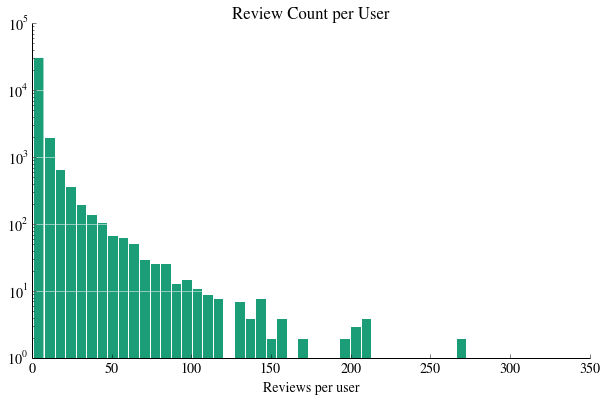In : #your code here brc=fulldf.groupby('business_id').review_id.count() ax=brc.hist(bins=50, log=True) remove_border(ax) plt.xlabel("Reviews per restaurant") plt.grid(False) plt.grid(axis = 'y', color ='white', linestyle='-') plt.title("Review Count per Restaurant");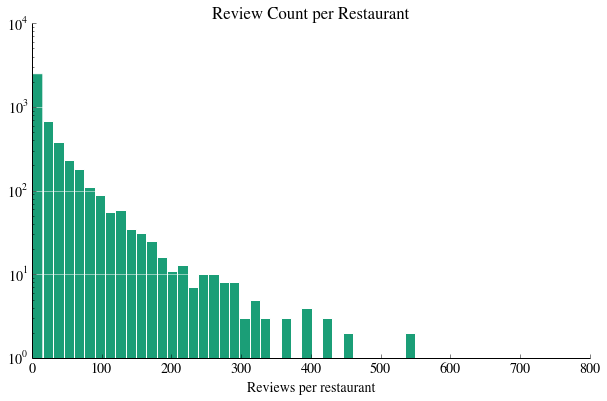In : #your code here print "Number of Reviews",fulldf.shape print "Number of Users", fulldf.user_id.unique().shape, "Number of Businesses", fulldf.business_id.unique().shape  Number of Reviews 149319 Number of Users 34789 Number of Businesses 4503  your answer here: There are more users than businesses. 1.2 Compute the average rating of reviews in the data set and a histogram of all the ratings in the dataset. In : #your code here print "Mean stars over all reviews:",fulldf.stars.mean() stars=fulldf.stars ax=stars.hist(bins=5) remove_border(ax) plt.xlabel("Star rating") plt.grid(False) plt.grid(axis = 'y', color ='white', linestyle='-') plt.title("Star ratings over all reviews");  Mean stars over all reviews: 3.74141268023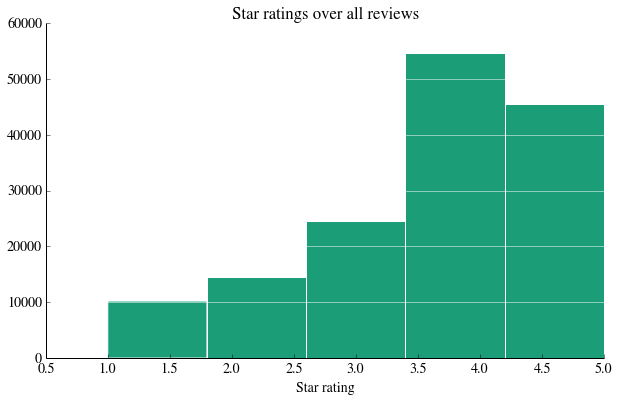The following function is used to re-compute review counts and averages whenever you subset a reviews data frame. We'll use it soon to construct a smaller, more computationally tractable data frame. In : def recompute_frame(ldf): """ takes a dataframe ldf, makes a copy of it, and returns the copy with all averages and review counts recomputed this is used when a frame is subsetted. """ ldfu=ldf.groupby('user_id') ldfb=ldf.groupby('business_id') user_avg=ldfu.stars.mean() user_review_count=ldfu.review_id.count() business_avg=ldfb.stars.mean() business_review_count=ldfb.review_id.count() nldf=ldf.copy() nldf.set_index(['business_id'], inplace=True) nldf['business_avg']=business_avg nldf['business_review_count']=business_review_count nldf.reset_index(inplace=True) nldf.set_index(['user_id'], inplace=True) nldf['user_avg']=user_avg nldf['user_review_count']=user_review_count nldf.reset_index(inplace=True) return nldf  1.3 Create a smaller data set in dataframe smalldf by looking for those businesses with more than 150 reviews and those users with more than 60 reviews. Include all the columns that were there in the parent dataframe. Since you have created a subset of the data set, use the method provided above to recalculate the averages. Print the number of unique users and items in this data set. Note that while this cut makes sure we have prolific users, the cut on businesses restores sparsity by reducing the number of reviews per user. In : #your code here smallidf=fulldf[(fulldf.user_review_count > 60) & (fulldf.business_review_count > 150)] smalldf=recompute_frame(smallidf)  How does this compare to the parent data set, in terms of size and sparsity? Once again, plot histograms of the review count grouped by user, and by the review count grouped by business, respectively, and describe the results In : #your code here print "Total Number of Reviews", smalldf.shape print "Users in this set", smalldf.user_id.unique().shape, "Restaurants",smalldf.business_id.unique().shape plt.figure() ax=smalldf.groupby('user_id').review_id.count().hist() remove_border(ax) plt.xlabel("Reviews per user") plt.grid(False) plt.grid(axis = 'y', color ='white', linestyle='-') plt.figure() ax=smalldf.groupby('business_id').review_id.count().hist() remove_border(ax) plt.xlabel("Reviews per restaurant") plt.grid(False) plt.grid(axis = 'y', color ='white', linestyle='-')  Total Number of Reviews 6165 Users in this set 240 Restaurants 172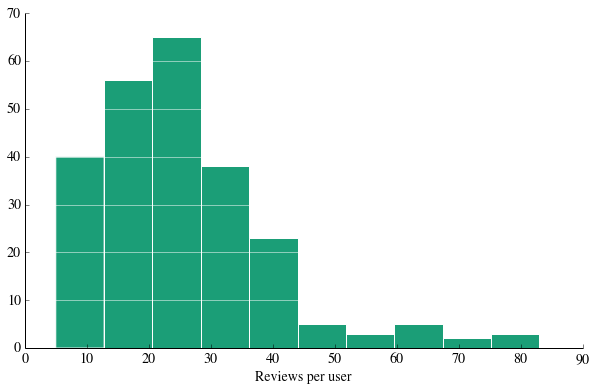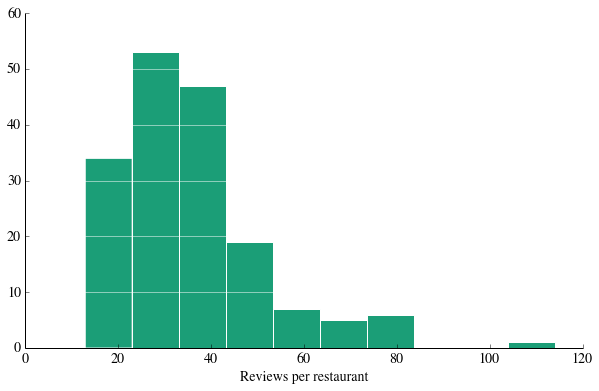your answer here: As we made a very specific cut on the data set, making sure we had those businesses with greater than 150 reviews, and those users with more than 60 reviews, this data set is not as sparse as the full one, with a range in both graphs where the histogram is somewhat flat rather than steeply declining. 1.4 Compute histograms of the average user rating in the smaller data set, and the average business rating in the smaller data set. Print the overall mean. In : #your code here plt.figure() avg_ratings_by_user=smalldf.groupby('user_id').stars.mean() ax=avg_ratings_by_user.hist() remove_border(ax) plt.xlabel("Average review score") plt.grid(False) plt.grid(axis = 'y', color ='white', linestyle='-') plt.title("Average User Rating") plt.figure() avg_ratings_by_biz=smalldf.groupby('business_id').stars.mean() ax=avg_ratings_by_biz.hist() remove_border(ax) plt.xlabel("Average review score") plt.grid(False) plt.grid(axis = 'y', color ='white', linestyle='-') plt.title("Average Restaurant Rating") plt.figure() print smalldf.stars.mean() plt.figure()  3.86763990268  Out: <matplotlib.figure.Figure at 0x107bcf150>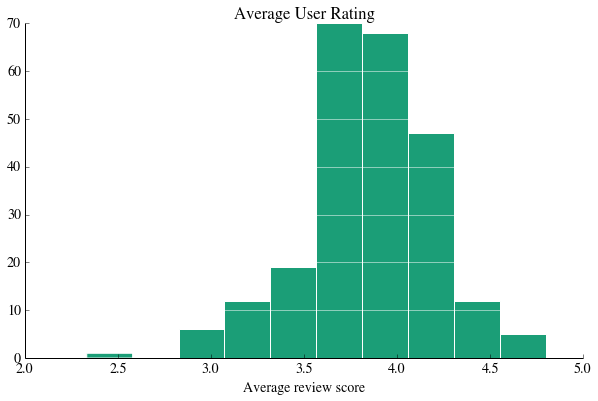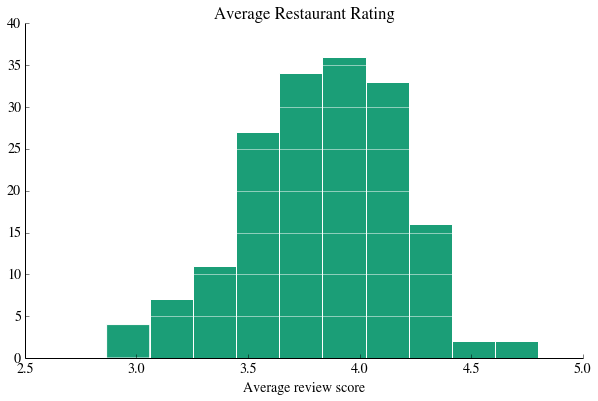<matplotlib.figure.Figure at 0x107869ad0> <matplotlib.figure.Figure at 0x107bcf150> ### Common Support¶ Lets now make a histogram of the common user support (the number of common reviewers) of each pair of restaurants on the smaller set, and print the mean. Pay attention to the code, as you will use parts of it later. (This code takes a bit of time to run, so be patient). The common support is an important concept, as for each pair of restaurants, its the number of people who reviewed both. It will be used to modify similarity between restaurants. If the common support is low, the similarity is less believable. In : restaurants=smalldf.business_id.unique() supports=[] for i,rest1 in enumerate(restaurants): for j,rest2 in enumerate(restaurants): if i < j: rest1_reviewers = smalldf[smalldf.business_id==rest1].user_id.unique() rest2_reviewers = smalldf[smalldf.business_id==rest2].user_id.unique() common_reviewers = set(rest1_reviewers).intersection(rest2_reviewers) supports.append(len(common_reviewers)) print "Mean support is:",np.mean(supports) plt.hist(supports)  Mean support is: 6.84679722562  Out: (array([ 7.02000000e+03, 4.98700000e+03, 1.79400000e+03, 5.90000000e+02, 1.95000000e+02, 7.60000000e+01, 2.20000000e+01, 1.00000000e+01, 1.00000000e+01, 2.00000000e+00]), array([ 0. , 5.1, 10.2, 15.3, 20.4, 25.5, 30.6, 35.7, 40.8, 45.9, 51. ]), <a list of 10 Patch objects>)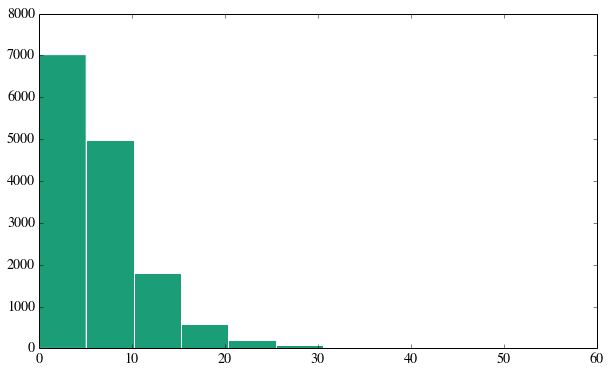As you can see, even though we chose a subset of the dataframe in which every restaurant had 150 reviews and every user had atleast made 60, the common support of most pairs of restaurants is really low, indeed less than 10!. ### Calculating Similarity¶ Users rate restaurants on a scale of 1-5. Even though this rating is integer valued, for the purposes of this assignment we shall treat it as a real number. Even though each reviewer uses the same 5-star scale when rating restaurants, comparing two users by comparing their raw user ratings can be problematic. Consider a user whose average rating is 2. This is a curmudgeonly user. Consider another whose average rating is 4. This is a rather enthusiastic one. How should we compare a 3 rating by the curmudgeonly one to a 5 rating of the enthusiastic one? It is for this purpose that we must subtract the average rating of the user from the actual rating of the restaurants in computing the similarity of two restaurants. This makes the above ratings by the two users comparable. We do this in the function pearson_sim defined below. If there is no common support (n_common=0), we have no basis for making a similarity estimate, and so we set the similarity to 0. In the case that the individual restaurant rating variance is 0, such as in the case where there is only one common reviewer (n_common=1), we return the NaN that the scipy pearsonr returns. We will deal with it soon, In : from scipy.stats.stats import pearsonr def pearson_sim(rest1_reviews, rest2_reviews, n_common): """ Given a subframe of restaurant 1 reviews and a subframe of restaurant 2 reviews, where the reviewers are those who have reviewed both restaurants, return the pearson correlation coefficient between the user average subtracted ratings. The case for zero common reviewers is handled separately. Its ok to return a NaN if any of the individual variances are 0. """ if n_common==0: rho=0. else: diff1=rest1_reviews['stars']-rest1_reviews['user_avg'] diff2=rest2_reviews['stars']-rest2_reviews['user_avg'] rho=pearsonr(diff1, diff2) return rho  The function get_restaurant_reviews defined below takes a restaurant business_id and a set of users, and returns the reviews of that restaurant by those users. You will use this function in calculating a similarity function, in 1.5. In : def get_restaurant_reviews(restaurant_id, df, set_of_users): """ given a resturant id and a set of reviewers, return the sub-dataframe of their reviews. """ mask = (df.user_id.isin(set_of_users)) & (df.business_id==restaurant_id) reviews = df[mask] reviews = reviews[reviews.user_id.duplicated()==False] return reviews  1.5 Write a function calculate_similarity that operates between two restaurants and calculates a similarity for them, taking a dataframe and a similarity function similarity_func. An example of the similarity_func is the pearson_sim we defined above. calculate_similarity operates as follows: 1. For each of the two restaurants, get the set of reviewers who have reviewed the restaurant and compute the intersection of these two sets. Also compute the number of common reviewers n_common. 2. Use the function get_restaurant_reviews defined below to get the reviews for each restaurant as made by these common reviewers. Notice that get_restaurant_reviews returns a sub data frame of reviews. 3. Calculate the similarity using similarity_func which takes the two reviews dataframes from part 2 and the number of common reviewers n_common as arguments 4. Return the similarity and n_common in a tuple (sim, n_common). If the similarity is a NaN, set the similarity to 0. In : """ Function -------- calculate_similarity Parameters ---------- rest1 : string The id of restaurant 1 rest2 : string The id of restaurant 2 df : DataFrame A dataframe of reviews, such as the smalldf above similarity_func : func A function like pearson_sim above which takes two dataframes of individual restaurant reviews made by a common set of reviewers, and the number of common reviews. This function returns the similarity of the two restaurants based on the common reviews. Returns -------- A tuple The first element of the tuple is the similarity and the second the common support n_common. If the similarity is a NaN, set it to 0 """ #your code here def calculate_similarity(rest1, rest2, df, similarity_func): # find common reviewers rest1_reviewers = df[df.business_id==rest1].user_id.unique() rest2_reviewers = df[df.business_id==rest2].user_id.unique() common_reviewers = set(rest1_reviewers).intersection(rest2_reviewers) n_common=len(common_reviewers) #get reviews rest1_reviews = get_restaurant_reviews(rest1, df, common_reviewers) rest2_reviews = get_restaurant_reviews(rest2, df, common_reviewers) sim=similarity_func(rest1_reviews, rest2_reviews, n_common) if np.isnan(sim): return 0, n_common return sim, n_common  ### Making a database of similarities¶ We now move to calculating a global database of pairwise restaurant similarities. We provide you here with a function to make a database of the similarities for each pair of restaurants in the database. The class Database is initialized in its constructor by taking as arguments a dataframe of reviews. The method populate_by calculating iterates over every possible pair of business_id's in the dataframe and populates the database with similarities and common supports. It takes as arguments a function the similarity function similarity_func like pearson_sim (calculate_similarity then uses this to calculate the similarity). The get method on the database can be used to retrieve the similarity for two business ids. (See Thu Oct 17th's class video for information about classes) In : class Database: "A class representing a database of similaries and common supports" def __init__(self, df): "the constructor, takes a reviews dataframe like smalldf as its argument" database={} self.df=df self.uniquebizids={v:k for (k,v) in enumerate(df.business_id.unique())} keys=self.uniquebizids.keys() l_keys=len(keys) self.database_sim=np.zeros([l_keys,l_keys]) self.database_sup=np.zeros([l_keys, l_keys], dtype=np.int) def populate_by_calculating(self, similarity_func): """ a populator for every pair of businesses in df. takes similarity_func like pearson_sim as argument """ items=self.uniquebizids.items() for b1, i1 in items: for b2, i2 in items: if i1 < i2: sim, nsup=calculate_similarity(b1, b2, self.df, similarity_func) self.database_sim[i1][i2]=sim self.database_sim[i2][i1]=sim self.database_sup[i1][i2]=nsup self.database_sup[i2][i1]=nsup elif i1==i2: nsup=self.df[self.df.business_id==b1].user_id.count() self.database_sim[i1][i1]=1. self.database_sup[i1][i1]=nsup def get(self, b1, b2): "returns a tuple of similarity,common_support given two business ids" sim=self.database_sim[self.uniquebizids[b1]][self.uniquebizids[b2]] nsup=self.database_sup[self.uniquebizids[b1]][self.uniquebizids[b2]] return (sim, nsup)  Lets run make_database and store the result in the global variable db. Lets print out an example entry. Running this function will take a bit of time. In : db=Database(smalldf) db.populate_by_calculating(pearson_sim)  In : db.get("z3yFuLVrmH-3RJruPEMYKw", "zruUQvFySeXyEd7_rQixBg")  Out: (0.39904554525734559, 7) ### K-Nearest restaurants (in similarity)¶ We are now going to find the k-nearest restaurants to a given restaurant based on the database of similarities that we calculated. But we have a problem. Consider the two cases where there is just one common reviewer, and where there are 40. In the former case, we might get a artificially high similarity based on the tastes of just this user, and thus we must reduce its importance in the nearest-neighbor calculation. In the latter case, we would get a much more unbiased estimator of the similarity of the two restaurants. To control the effect of small common supports, we can shrink our pearson co-efficients. We shall do this by using the "regularization" parameter reg: $$s_{mj} = \frac{N_{common}\, \rho_{mj}}{N_{common}+reg}$$ where$N_{common}$(n_common) is the common reviewer support and$\rho_{ij}$is the pearson co-relation coefficient. Recall the notions of regularization introduced in class. We want to reduce the variance in our estimates, so we pull our estimates in toward a conservative point in a way that strongly corrals in estimates when there is very little data, but allows the data to speak when there is a lot. This can be shown as equivalent to adding in a reg amount of bayesian prior, as Joe has alluded to in class. A good value of the regularizer is intuitively one that dosent affect the similarity when the common support is high ~ 10, but has a large effect when the support is small. In this case, values of 2-4 are good. Usually, the value of reg is determined using cross-validation, but for the sake of simplicity we will generally set it to 3. We define a function shrunk_sim which takes the sim and n_common obtained from the database, and shrinks the similarity down using the regularizer reg. In : def shrunk_sim(sim, n_common, reg=3.): "takes a similarity and shrinks it down by using the regularizer" ssim=(n_common*sim)/(n_common+reg) return ssim  1.6 Now we can move to writing a knearest function, which finds the k nearest neighbors of a given restaurant based on the shrunk similarities we calculate. Note that as defined here, the nearest neighbors are global over the entire set of restaurants, as opposed to being restricted to the restaurants a user has reviewed(we shall do that in the next problem). Thus, this is an expensive function! Write a knearest that returns a k-length sorted list of 3-tuples each corresponding to a restaurant. The tuple structure is (business_id, shrunken similarity score, common support) where the similarity score and common support are with respect to the restaurant whose neighbors we are finding, and the business_id is the id of the "nearby" restaurant found. The nearby restaurants are found from a supplied numpy array of restaurants set_of_restaurants. The spec for the function is given below. HINT: use itemgetter from the operator module to do the sorting. In : """ Function -------- knearest Parameters ---------- restaurant_id : string The id of the restaurant whose nearest neighbors we want set_of_restaurants : array The set of restaurants from which we want to find the nearest neighbors dbase : instance of Database class. A database of similarities, on which the get method can be used to get the similarity of two businessed. e.g. dbase.get(rid1,rid2) k : int the number of nearest neighbors desired, default 7 reg: float the regularization. Returns -------- A sorted list of the top k similar restaurants. The list is a list of tuples (business_id, shrunken similarity, common support). """ #your code here from operator import itemgetter def knearest(restaurant_id, set_of_restaurants, dbase, k=7, reg=3.): """ Given a restaurant_id, dataframe, and database, get a sorted list of the k most similar restaurants from the entire database. """ similars=[] for other_rest_id in set_of_restaurants: if other_rest_id!=restaurant_id: sim, nc=dbase.get(restaurant_id, other_rest_id) ssim=shrunk_sim(sim, nc, reg=reg) similars.append((other_rest_id, ssim, nc )) similars=sorted(similars, key=itemgetter(1), reverse=True) return similars[0:k]  ### Ok it's time to recommend!¶ Lets choose the two very different businesses in the dataframe In : testbizid="eIxSLxzIlfExI6vgAbn2JA" testbizid2="L-uPZxooP_ziXCtRrWi8Pw"  We provide functions to look up a business name given a business id, and a username given a user id. In : def biznamefromid(df, theid): return df['biz_name'][df['business_id']==theid].values def usernamefromid(df, theid): return df['user_name'][df['user_id']==theid].values  In : print testbizid, biznamefromid(smalldf,testbizid) print testbizid2, biznamefromid(smalldf, testbizid2)  eIxSLxzIlfExI6vgAbn2JA Lobbys Beef Burgers Dogs L-uPZxooP_ziXCtRrWi8Pw Café Monarch  #### Get top matches¶ Its now time to answer the question: "if you liked this, you might also like these". We use our testbizid and testbizid2 to compute the k=7 nearest neighbors with a regularization of 3. . We print these top 7 matches names, along with their similarity coefficient and common support. In : tops=knearest(testbizid, smalldf.business_id.unique(), db, k=7, reg=3.) print "For ",biznamefromid(smalldf, testbizid), ", top matches are:" for i, (biz_id, sim, nc) in enumerate(tops): print i,biznamefromid(smalldf,biz_id), "| Sim", sim, "| Support",nc  For Lobbys Beef Burgers Dogs , top matches are: 0 La Condesa Gourmet Taco Shop | Sim 0.598714448434 | Support 6 1 Citizen Public House | Sim 0.571428571429 | Support 4 2 FnB | Sim 0.527129890943 | Support 5 3 Defalco's Italian Grocery | Sim 0.519456555658 | Support 6 4 Republic Ramen + Noodles | Sim 0.519140146937 | Support 5 5 unPhogettable | Sim 0.5 | Support 3 6 Haus Murphy's | Sim 0.467637235308 | Support 3  In : tops2=knearest(testbizid2, smalldf.business_id.unique(), db, k=7, reg=3.) print "For ",biznamefromid(smalldf, testbizid2), ", top matches are:" for i, (biz_id, sim, nc) in enumerate(tops2): print i,biznamefromid(smalldf,biz_id), "| Sim", sim, "| Support",nc  For Café Monarch , top matches are: 0 Postino Arcadia | Sim 0.625 | Support 5 1 The Main Ingredient Ale House And Café | Sim 0.571428571429 | Support 4 2 Brio Tuscan Grille | Sim 0.571428571429 | Support 4 3 Kazimierz World Wine Bar | Sim 0.5 | Support 3 4 Harlow's Cafe | Sim 0.5 | Support 3 5 The Fry Bread House | Sim 0.5 | Support 3 6 Cien Agaves Tacos & Tequila | Sim 0.5 | Support 3  We can see that these two restaurants are in somewhat different orbits :-). Lets now turn our attention to another question: what are the top recommendations for a user? To answer this we must find the user's top rated restaurants, find the nearest neighbors of these restaurants, merge these lists while removing the duplicates and the ones that the user has already rated, and sort by the restaurant's average rating. We provide the code to get the user's top choices in a subset data frame. In : def get_user_top_choices(user_id, df, numchoices=5): "get the sorted top 5 restaurants for a user by the star rating the user gave them" udf=df[df.user_id==user_id][['business_id','stars']].sort(['stars'], ascending=False).head(numchoices) return udf testuserid="7cR92zkDv4W3kqzii6axvg" print "For user", usernamefromid(smalldf,testuserid), "top choices are:" bizs=get_user_top_choices(testuserid, smalldf)['business_id'].values [biznamefromid(smalldf, biz_id) for biz_id in bizs]  For user Vern top choices are:  Out: ['Local Breeze', "Carly's Bistro", 'Tee Pee Mexican Food', 'District American Kitchen and Wine Bar', 'Los Reyes de la Torta'] #### Get top recommendations for user.¶ 1.7 Its your job now to write a function get_top_recos_for_user which takes as arguments a userid, the n top choices for the user, the dataframe, k, and a regularizer, and returns the top recommendations obtained from combining the restaurants that are neighbors of each of the n choices, in the way described in the previous paragraph. This returned list is a list of tuples (restaurant_id, business_avg) sorted by business_avg where business_avg is the average rating of the restaurant over the dataframe. In : """ Function -------- get_top_recos_for_user Parameters ---------- userid : string The id of the user for whom we want the top recommendations df : Dataframe The dataframe of restaurant reviews such as smalldf dbase : instance of Database class. A database of similarities, on which the get method can be used to get the similarity of two businesses. e.g. dbase.get(rid1,rid2) n: int the n top choices of the user by star rating k : int the number of nearest neighbors desired, default 8 reg: float the regularization. Returns -------- A sorted list of the top recommendations. The list is a list of tuples (business_id, business_avg). You are combining the k-nearest recommendations for each of the user's n top choices, removing duplicates and the ones the user has already rated. """ #your code here def get_top_recos_for_user(userid, df, dbase, n=5, k=7, reg=3.): bizs=get_user_top_choices(userid, df, numchoices=n)['business_id'].values rated_by_user=df[df.user_id==userid].business_id.values tops=[] for ele in bizs: t=knearest(ele, df.business_id.unique(), dbase, k=k, reg=reg) for e in t: if e not in rated_by_user: tops.append(e) #there might be repeats. unique it ids=[e for e in tops] uids={k:0 for k in list(set(ids))} topsu=[] for e in tops: if uids[e] == 0: topsu.append(e) uids[e] =1 topsr=[] for r, s,nc in topsu: avg_rate=df[df.business_id==r].stars.mean() topsr.append((r,avg_rate)) topsr=sorted(topsr, key=itemgetter(1), reverse=True) if n < len(topsr): return topsr[0:n] else: return topsr  Lets print the top recommendations for testuserid, with a regularization of 3. In : print "For user", usernamefromid(smalldf,testuserid), "the top recommendations are:" toprecos=get_top_recos_for_user(testuserid, smalldf, db, n=5, k=7, reg=3.) for biz_id, biz_avg in toprecos: print biznamefromid(smalldf,biz_id), "| Average Rating |", biz_avg  For user Vern the top recommendations are: Rokerij | Average Rating | 4.37931034483 Wildfish Seafood Grille | Average Rating | 4.29411764706 Cornish Pasty Company | Average Rating | 4.20689655172 Pappadeaux Seafood Kitchen | Average Rating | 4.18518518519 Four Peaks Brewing Co | Average Rating | 4.16666666667  ## Problem 2: A user based recommender with predicted ratings¶ This is all very nice. We can provide ratings based on global similarities to a restaurant. However, in many cases this is not enough. For example, it is hard to judge if the above recommendations are any good. In the usual testing paradigm, say that we break the dataframe into train and test. Based on the training set, I am recommended restaurant B. Now, I have rated B, but that information is in the testing set. I have no way of comparing the rating I give B in the testing set, to the similarity computed from the training set that was used to make the recomendation. The best I could do is to compare the average rating of restaurant B in the training set to my rating of restaurant B in the test set. In this section, we shift our focus to more fine-grained predictions about each user, and try to predict what rating a user would give to a restaurant they have never tried before. To do this, we will try to personalize the information we use even further, and only pool information from restaurants that the user has rated. This allows us to return to the original problem of prediction$Y_{um}$for a restaurant$m$that user$u$has never rated before. Using our newly computed similarity metrics, we can modify our original baseline estimate by pulling in information from the user's neighborhood of the restaurant$m$, and predict$Y_{um}$as: $$\hat{Y_{um}} = \hat Y^{baseline}_{um}\, + \,\frac{\sum\limits_{j \in S^{k}(m;u)} s_{mj} ( Y_{uj} - \hat Y^{baseline}_{uj} )}{\sum\limits_{j \in S^{k}(m;u)} s_{mj} }$$ where$s^{k}(m;u)$is the$k$neighbor items of item$m$which have been rated by user$u$. Now, this is not a particularly good assumption, especially in the situation where a restaurant is new (new item problem) or a user is new (cold start problem), or in the case when there are very few reviewers of a restaurant, or very few reviews by a user respectively. However, one must start somewhere! Notice that in adding in the similarity term, we subtract the baseline estimate from the observed rating of the user's neighbor items. ### Defining the predicted rating¶ 2.1 Write a function knearest_amongst_userrated, analogous to the knearest function we defined above, to find the nearest k neighbors to a given restaurant from the restaurants that the user has already rated. This function will take as arguments the restaurant_id, the user_id, the dataframe of reviews, the database, the k, and the regularizer reg. Just like before, return a k-length sorted list of 3-tuples each corresponding to a restaurant. HINT: use the knearest function you defined earlier In : """ Function -------- knearest_amongst_userrated Parameters ---------- restaurant_id : string The id of the restaurant whose nearest neighbors we want user_id : string The id of the user, in whose reviewed restaurants we want to find the neighbors df: Dataframe The dataframe of reviews such as smalldf dbase : instance of Database class. A database of similarities, on which the get method can be used to get the similarity of two businessed. e.g. dbase.get(rid1,rid2) k : int the number of nearest neighbors desired, default 7 reg: float the regularization. Returns -------- A sorted list of the top k similar restaurants. The list is a list of tuples (business_id, shrunken similarity, common support). """ #your code here def knearest_amongst_userrated(restaurant_id, user_id, df, dbase, k=7, reg=3.): dfuser=df[df.user_id==user_id] bizsuserhasrated=dfuser.business_id.unique() return knearest(restaurant_id, bizsuserhasrated, dbase, k=k, reg=reg)  2.2 Now write a function that returns the predicted rating for a user and an item using the formula at the beginning of this problem. Include code to deal with the possibility that the sum of scores that goes in the denominator is 0: return an predicted rating of the baseline portion of the formula in that case. This function rating takes as arguments the dataframe, the database, the wanted restaurant_id and user_id, and k as well as the regularizer. In : """ Function -------- rating Parameters ---------- df: Dataframe The dataframe of reviews such as smalldf dbase : instance of Database class. A database of similarities, on which the get method can be used to get the similarity of two businessed. e.g. dbase.get(rid1,rid2) restaurant_id : string The id of the restaurant whose nearest neighbors we want user_id : string The id of the user, in whose reviewed restaurants we want to find the neighbors k : int the number of nearest neighbors desired, default 7 reg: float the regularization. Returns -------- A float which is the impued rating that we predict that user_id will make for restaurant_id """ #your code here def rating(df, dbase, restaurant_id, user_id, k=7, reg=3.): mu=df.stars.mean() users_reviews=df[df.user_id==user_id] nsum=0. scoresum=0. nears=knearest_amongst_userrated(restaurant_id, user_id, df, dbase, k=k, reg=reg) restaurant_mean=df[df.business_id==restaurant_id].business_avg.values user_mean=users_reviews.user_avg.values scores=[] for r,s,nc in nears: scoresum=scoresum+s scores.append(s) r_reviews_row=users_reviews[users_reviews['business_id']==r] r_stars=r_reviews_row.stars.values r_avg=r_reviews_row.business_avg.values rminusb=(r_stars - (r_avg + user_mean - mu)) nsum=nsum+s*rminusb baseline=(user_mean +restaurant_mean - mu) #we might have nears, but there might be no commons, giving us a pearson of 0 if scoresum > 0.: val = nsum/scoresum + baseline else: val=baseline return val  For the top-recommendations in the variable toprecos from the previous section, we compute the predicted rating and compare it with the average rating over all users available inside the tuples that make up toprecos. We use a k of 7 and regularization 3. For comparision we also print this users' average rating. Do you notice anything interesting about how the order has changed from when we did this with the global similarities? (for you to think, not to answer) In : print "User Average", smalldf[smalldf.user_id==testuserid].stars.mean(),"for",usernamefromid(smalldf,testuserid) print "Predicted ratings for top choices calculated earlier:" for biz_id,biz_avg in toprecos: print biznamefromid(smalldf, biz_id),"|",rating(smalldf, db, biz_id, testuserid, k=7, reg=3.),"|","Average",biz_avg  User Average 3.5652173913 for Vern Predicted ratings for top choices calculated earlier: Rokerij | 4.71714023074 | Average 4.37931034483 Wildfish Seafood Grille | 4.27594504172 | Average 4.29411764706 Cornish Pasty Company | 4.62810510121 | Average 4.20689655172 Pappadeaux Seafood Kitchen | 4.08845573953 | Average 4.18518518519 Four Peaks Brewing Co | 4.26174734161 | Average 4.16666666667  ### Testing the ratings¶ Let us compare the predicted ratings with a user's ratings. Note that we are doing this on the same set that we constructed the predictions with, so this is not a validation of the procedure, but simply a check of the procedure's fit. We first write a helper function to return the user score for a restaurant, and the restaurant's average score over all users. In : def get_other_ratings(restaurant_id, user_id, df): "get a user's rating for a restaurant and the restaurant's average rating" choice=df[(df.business_id==restaurant_id) & (df.user_id==user_id)] users_score=choice.stars.values average_score=choice.business_avg.values return users_score, average_score  For the user testuserid, we loop over the variable bizs (which is a set of restaurants the user has rated) and print the predicted rating, and the actual rating and restaurant average rating obtained using the function above. We again use k=7 and a regularization of 3. In : print "for user",usernamefromid(smalldf,testuserid), 'avg', smalldf[smalldf.user_id==testuserid].stars.mean() for biz_id in bizs: print "----------------------------------" print biznamefromid(smalldf, biz_id) print "Predicted Rating:",rating(smalldf, db, biz_id, testuserid, k=7, reg=3.) u,a=get_other_ratings(biz_id, testuserid, smalldf) print "Actual User Rating:",u,"Avg Rating",a  for user Vern avg 3.5652173913 ---------------------------------- Local Breeze Predicted Rating: 4.2280987611 Actual User Rating: 5 Avg Rating 4.0 ---------------------------------- Carly's Bistro Predicted Rating: 3.99008654065 Actual User Rating: 5 Avg Rating 3.5 ---------------------------------- Tee Pee Mexican Food Predicted Rating: 3.52640184162 Actual User Rating: 5 Avg Rating 3.04347826087 ---------------------------------- District American Kitchen and Wine Bar Predicted Rating: 3.80281696528 Actual User Rating: 4 Avg Rating 3.55263157895 ---------------------------------- Los Reyes de la Torta Predicted Rating: 3.41514298977 Actual User Rating: 4 Avg Rating 4.13157894737  2.3 Explain in words why the predicted ratings are lower than the actual ratings. How do the user average rating and restaurant average rating affect this? How does sparsity affect the predicted ratings? your answer here Recall that bizs (defined just above question 1.7) has restaurants sorted by Vern's actual star ratings. This means that in this sample, we are looking at Vern's top rated restaurants. The predicted ratings are lower because these are Vern's top 5 choices, which represent the largest positive deviations away from Vern's mean rating of 3.57. Because we are looking at the upper tail of Vern's rating distribution, but pooling information together from the K nearest neighbors among Vern's rated restaurants to construct the predicted rating, the predicted ratings should fall closer to Vern's user mean than the true ones do. Taking into account the average restaurant rating helps a little bit here because we can adjust the predicted rating to reflect an overall very good restaurant, but it does not counteract the effect of looking at the upper tail of Vern's ratings. Note that if we were to take Vern's bottom 5 restaurants, we would see the opposite effect. In general, the larger K is (assuming that the similarities within this neighborhood are positive), the closer the predicted rating will be to Vern's user average (this is the bias limit in the bias-variance tradeoff). Similarly, the smaller K is, the more likely we are to have user ratings that are close to the observed rating (the variance limit). The sparsity of the data affects how quickly we move from the variance limit to the bias limit as we increase K. If there were a lot of very similar restaurants in the dataset that Vern had ranked very highly, even with K relatively large, it would be possible to see a predicted rating much closer to the extremely positive ratings we see here in Vern's top 5 (see the results in question 4.4). As these data are now, however, even the most similar 7 restaurants to these that Vern rated so highly lie closer to Vern's mean. ### Error Analysis¶ This next function takes a set of actual ratings, and a set of predicted ratings, and plots the latter against the former. We can use a graph of this kind to see how well or badly we do in our predictions. Since the nearest neighbor models can have alternating positive and negative similarities (the sum of similarity weights in the denominator can get large), the ratings can get very large. Thus we restrict ourselves to be between -10 and 15 in our ratings and calculate the fraction within these bounds. We also plot the line with unit slope, line sehments joining the means, and a filled in area representing one standard deviation from the mean. The first argument to compare_results is a numpy array of the actual star ratings obtained from the dataframe, while the second argument is the numpy array of the predicted ones. (Feel free to improve this function for your display) In : def compare_results(stars_actual, stars_predicted, ylow=-10, yhigh=15, title=""): """ plot predicted results against actual results. Takes 2 arguments: a numpy array of actual ratings and a numpy array of predicted ratings scatterplots the predictions, a unit slope line, line segments joining the mean, and a filled in area of the standard deviations." """ fig=plt.figure() df=pd.DataFrame(dict(actual=stars_actual, predicted=stars_predicted)) ax=plt.scatter(df.actual, df.predicted, alpha=0.2, s=30, label="predicted") plt.ylim([ylow,yhigh]) plt.plot([1,5],[1,5], label="slope 1") xp=[1,2,3,4,5] yp=df.groupby('actual').predicted.mean().values plt.plot(xp,yp,'k', label="means") sig=df.groupby('actual').predicted.std().values plt.fill_between(xp, yp - sig, yp + sig, color='k', alpha=0.2) plt.xlabel("actual") plt.ylabel("predicted") plt.legend(frameon=False) remove_border() plt.grid(False) plt.title(title) print "fraction between -15 and 15 rating", np.mean(np.abs(df.predicted) < 15)  2.4 For each review in the data set, obtain a prediction from the entire dataframe smalldf. Use the function compare_results above to plot the predicted ratings against the observed ones. Make 4 such graphs, at k=3 and k=10, and for reg=3. and reg=15. Note that this analysis is not strictly a model check because we are testing on the training set. However, since the user averages would change each time a cross-validation split was done on the set, we would incur the prohibitive expense of redoing the database each time. This would be better done on a cluster, using map-reduce or other techniques. While we explore map-reduce later in this homework, we shall not do any cross-validation. Explain the results you get in the graphs in words. In : #your code here def make_results_plot(df,k,reg): uid=smalldf.user_id.values bid=smalldf.business_id.values actual=smalldf.stars.values predicted=np.zeros(len(actual)) counter=0 for user_id, biz_id in zip(uid,bid): predicted[counter]=rating(smalldf, db, biz_id, user_id, k=k, reg=reg) counter=counter+1 compare_results(actual, predicted)  In : #your code here print "k=3, reg=3." make_results_plot(smalldf,3,3.) plt.title("k=3, reg=3.") print "k=3, reg=15." make_results_plot(smalldf,3,15.,) plt.title("k=3, reg=15.") print "k=10, reg=3." make_results_plot(smalldf,10,3.) plt.title("k=10, reg=3.") print "k=10, reg=15." make_results_plot(smalldf,10,15.,) plt.title("k=10, reg=15.")  k=3, reg=3. fraction between -15 and 15 rating 1.0 k=3, reg=15. fraction between -15 and 15 rating 0.999837793998 k=10, reg=3. fraction between -15 and 15 rating 0.996431467964 k=10, reg=15. fraction between -15 and 15 rating 0.997080291971  Out: <matplotlib.text.Text at 0x10cec8250>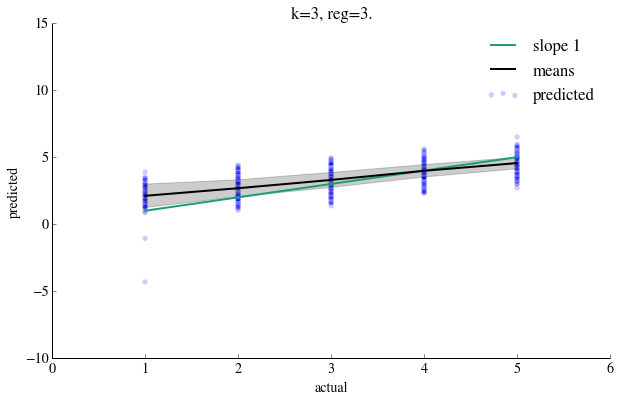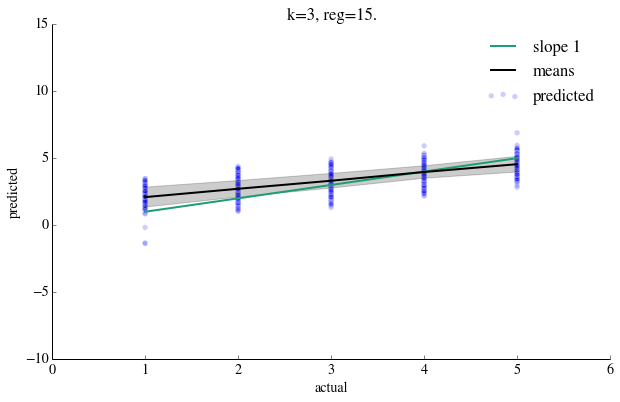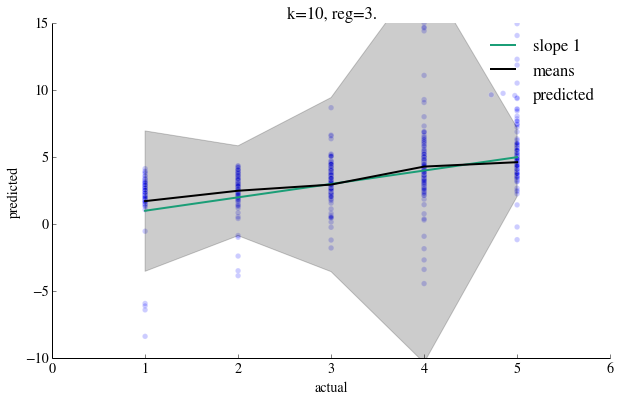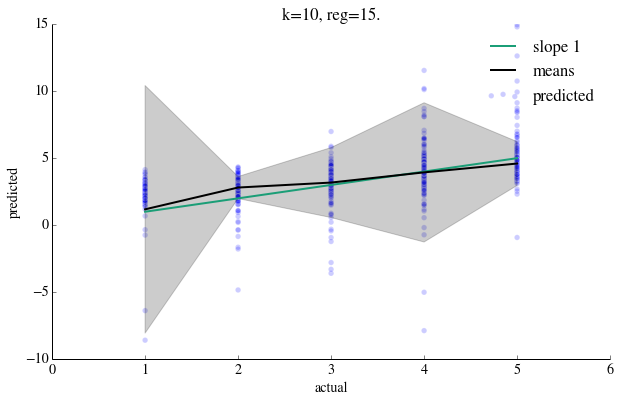your answer here If you are a bit confused by the look of these graphs, you should be! For k=3, the predicted values are quite well-behaved, with the exception of several predictions that have extremely large magnitudes. It appears that the predicted values are pulled into the mean star rating, which sits somewhere around 3.8, so ratings on the low end are overestimated, and similarly ratings on the high end underestimated. The regularization does not appear to have a strong effect when k = 3. For k=10, the predicted values are much less stable, with many more extreme predictions. The means appear to track better with the true means. The regularization has a much more extreme, although indirect, effect on the appearance of the plot. Since regularization has stronger effects on similarity scores between restaurants that have small common support, we can see that increasing k makes the predictions more sensitive to the regularization because in a small dataset, the common support between a restaurant and it's 10-nearest one will be quite small. Note that this example does not seem to follow the standard bias-variance tradeoff, where we would expect small k to give a unbiased estimates that capture the extremes, while we would expect large k to give biased estimates that pull extreme values toward the mean. A large reason for this failure for this example to capture this behavior is that we have defined similarity scores that can be positive or negative, and the bias-variance logic is based on the more standard setting where we average together values that have strictly positive weights. When you have negative weights, it's possible that the sum of$s_{ij}$'s in a neighborhood can get close to zero, making our estimator$\hat Y_{um}$explode in the positive or negative direction since this would entail dividing by (nearly) zero. Thus for those restaurants where the denominator goes to 0 (more likely to happen at larger k as you have more chances of it there being more weights to add), the ratings are unstable, even numerically! This problem is less pronounced in large datasets or with small k because the k-nearest restaurants are likely to have positive similarity with the current one. However, with small datasets, we can find that even with k relatively small (in this case, around 10), there are negative similarities in the k-neighborhood that make the estimator unstable. This sort of instability would be much less pronounced in the large dataset. If we were to rescale the similarities to be positive, say between 0 and 1, the behavior of the estimator with respect to$k$and$reg$would be quite different. (SEE BELOW!) ### IMPORTANT SOLUTION ADDENDUM¶ The wide wings we see above are in the error graph are due to small values of the denominator in the similarity sum. If you were writing a production recommender, using the ratings as is from Q2 would be a very bad idea. Indeed the very idea of nearest neighbor should engender in you the idea of distances; and distances should not be negative. Furthermore, you would not be able to use distances other than those implied by the correlation coefficient in the calculation of nearest neighbors (such as a Manhattan distance or Jacard similarity). We can fix this for the case of perarson coefficient by just a simple rescaling to the 0-1 range! $$\rho \rightarrow \frac{\rho+1}{2}$$ . This translates into changing the shrunk score: $$s \rightarrow \frac{s}{2} + \frac{f}{2}$$ where $$f = \frac{N_{common}}{N_{common}+reg}$$ Note that the new quantity is really an inverse distance, and thus we'll sort by its smallness as before. In : def knearest_pos(restaurant_id, set_of_restaurants, dbase, k=7, reg=3.): """ Given a restaurant_id, dataframe, and database, get a sorted list of the k most similar restaurants from the entire database. """ similars=[] for other_rest_id in set_of_restaurants: if other_rest_id!=restaurant_id: sim, nc=dbase.get(restaurant_id, other_rest_id) ssim=shrunk_sim(sim, nc, reg=reg) similars.append((other_rest_id, ssim/2.0 + float(nc)/(float(nc)+reg), nc )) similars=sorted(similars, key=itemgetter(1), reverse=True) return similars[0:k] def knearest_amongst_userrated_pos(restaurant_id, user_id, df, dbase, k=7, reg=3.): dfuser=df[df.user_id==user_id] bizsuserhasrated=dfuser.business_id.unique() return knearest_pos(restaurant_id, bizsuserhasrated, dbase, k=k, reg=reg)  In : def rating_pos(df, dbase, restaurant_id, user_id, k=7, reg=3.): mu=df.stars.mean() users_reviews=df[df.user_id==user_id] nsum=0. scoresum=0. nears=knearest_amongst_userrated_pos(restaurant_id, user_id, df, dbase, k=k, reg=reg) restaurant_mean=df[df.business_id==restaurant_id].business_avg.values user_mean=users_reviews.user_avg.values scores=[] for r,sold,nc in nears: s=sold/2.0 shrink_factor=float(nc)/(float(nc)+reg) s=s+shrink_factor/2.0 scoresum=scoresum+s scores.append(s) r_reviews_row=users_reviews[users_reviews['business_id']==r] r_stars=r_reviews_row.stars.values r_avg=r_reviews_row.business_avg.values rminusb=(r_stars - (r_avg + user_mean - mu)) nsum=nsum+s*rminusb baseline=(user_mean +restaurant_mean - mu) #we might have nears, but there might be no commons, giving us a pearson of 0 if scoresum > 0.: val = nsum/scoresum + baseline else: val=baseline return val  In : def make_results_plot_pos(df,k,reg): uid=smalldf.user_id.values bid=smalldf.business_id.values actual=smalldf.stars.values predicted=np.zeros(len(actual)) counter=0 for user_id, biz_id in zip(uid,bid): predicted[counter]=rating_pos(smalldf, db, biz_id, user_id, k=k, reg=reg) counter=counter+1 compare_results(actual, predicted, ylow=1, yhigh=5)  NOTICE: when comparing graphs note that the limits have changed to make the bias-variance comparison more visible. The regularizer is set to 1 to reduce the regularization to something small, rather than something optimal, as well. Also to make things more extreme, I choose k=15 rather than k=10. In : print "k=2, reg=1." make_results_plot_pos(smalldf,2,1.) plt.title("k=2, reg=1.") print "k=2, reg=15." make_results_plot_pos(smalldf,2,15.,) plt.title("k=2, reg=15.") print "k=15, reg=1." make_results_plot_pos(smalldf,15,1.) plt.title("k=15, reg=1.") print "k=15, reg=15." make_results_plot_pos(smalldf,15,15.,) plt.title("k=15, reg=15.")  k=2, reg=1. fraction between -15 and 15 rating 1.0 k=2, reg=15. fraction between -15 and 15 rating 1.0 k=15, reg=1. fraction between -15 and 15 rating 1.0 k=15, reg=15. fraction between -15 and 15 rating 1.0  Out: <matplotlib.text.Text at 0x10cef8990>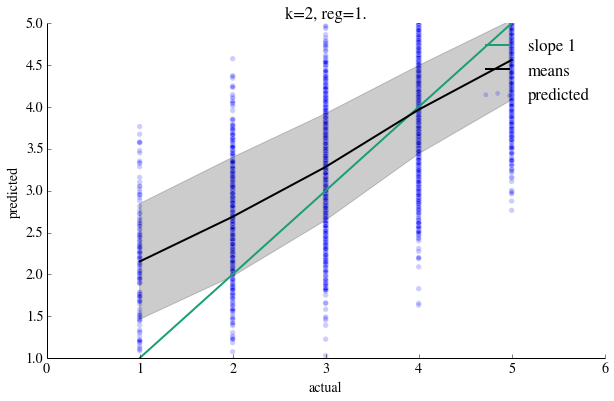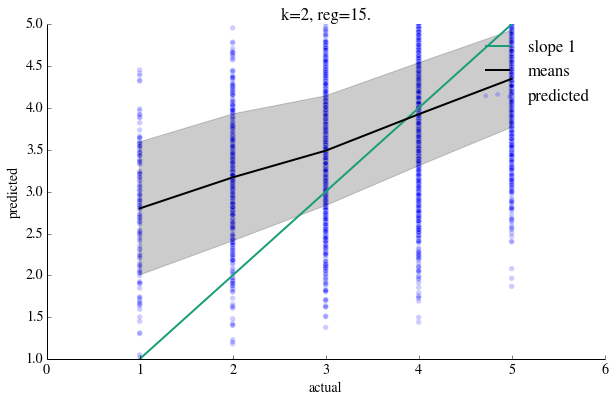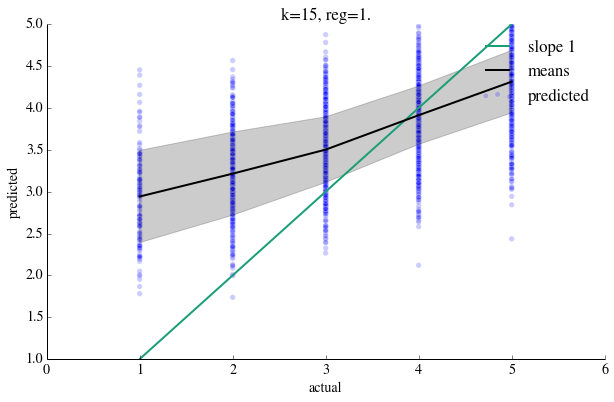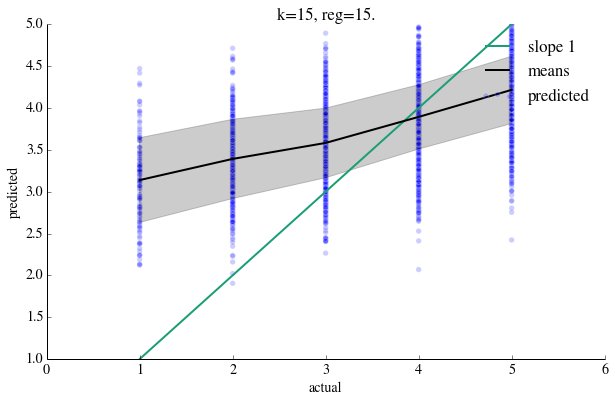Notice firstly, that at low regularization, at low k (variance limit), the predictions follow the green line more than at high k ( bias limit). This is, as mentioned earlier, due to the bias limit pulling the extreme groups of ratings in towards the mean of all the reviews. Note that increasing bias decreases the variation between the rating groups, resulting in a flatter black curve. The second thing to notice that as k goes up, the precision as measured by the width of the grey region (which measures the variance WITHIN rating groups, not between them) gets smaller. The third thing to notice is that adding in regularization in the variance (low k) limit actually increases the bias, which is not surprising as it tends to push things towards the center. In many machine learning situations this is a desirable thing, as it reduces overfitting. In the high bias limit, as expected, it does not have much of an impact. Note that regularization had more of an impact in the previous set of graphs, as it pushed similarity towards 0. One might ask: why not plot these graphs in the homework in the first place: using distances is the correct way to do it, and dosent lead to the subtraction problem. Its a good question, and you should use positive similarities in a production recommender. For the puposes of this homework, i think the k=10 graphs in the previous set illustrate the problem with sparsity in a much more dramatic way, leading to the "bellows" in the graph. However, in real life, there's no real justification for using similarities that can be negative as weights. If we think of KNN as a way of constructing local averages, you need those averages to be constructed using positive weights. 2.5 Outline a process, in words, for choosing the nearest neighbor parameter k. For this question fix the regularization parameter reg at 3. your answer here We could use$F$-fold cross-validation (usually called$k$-fold cross-validation, but we've already used$k$here to mean something else!). Specifically, we could randomly partition the data into$F$equally sized folds (pieces), making sure that each fold includes at least one rating for each restaurant and one rating by each user (it would probably best to make sure that if$K$were the maximum$k$you would be considering, that each user and each restaurant appear at least$K$times in each fold). For each value of$k$, and for each fold, we could repeat the procedure above for predicting user ratings by computing similarities using$F-1$of the folds to compute similarities and computing the prediction error in the held out fold. We could then choose the$k$with the smallest average prediction error across the folds, and recompute the recommender on the whole dataset using the chosen value of$k$. If we wanted to both choose a good value for$k$and check how well we could expect the result to generalize, we could divide the dataset into$F+1$folds, and keep the last fold out as a verification set. We could perform the cross-validation above on$F$folds to select$k$, then upon selecting$k$use all$F$folds to create a recommender, and see how well this recommender predicted ratings in the final validation set. ## Q3 Bayesian Chocolates: Model based recommendations¶ In this part of the homework, you will use your newly minted Bayesian and Gibbs sampler skills to write a recommender that uses Bayesian techniques to impute ratings. ### Model-Based Recommendations¶ #### A Note on Frequentist and Bayesian Procedures¶ In the previous section we implemented a procedure (a set of instructions for processing data) for giving recommendations and predicting user ratings for restaurants. This procedure involved a number of arbitrary choices -- for example, the particular measure of similarity between restaurants, or the weighting scheme for constructing a predicted rating. It also gave no sense of uncertainty -- in the case of giving recommendations, there was no statement about how we would expect the ranking from the procedure to compare to the user's true opinions of restaurants, and in the case of predicting ratings, there was no confidence interval for the prediction. It is possible in repeated applications of the above procedure to see how it performs in the long run. Based on this long-run performance we could potentially justify certain functional choices and compute measurements of uncertainty. This framework of proposing a procedure first, then evaluating its performance in real or hypothetical replications of the experiment is an example of a frequentist approach to a problem. One aspect of the frequentist approach is that the proposed procedure does not necessarily have to be derived from a model (although it often is). While this means that a proposed procedure may be more flexible or robust than a model-based procedure, it also means that there is no natural way to justify certain functional choices or construct uncertainty estimates. In contrast, the Bayesian approach to a problem always begins with a probablistic model for how the data were generated. Assuming this model is true, the posterior distribution over unknown quantities (either parameters to be estimated or unobserved data to be predicted) gives a single coherent expression of what the observed data tell us about the unknowns. By summarizing the posterior distribution, we can derive the exact functional form of a procedure for constructing estimates or predictions. We call a procedure derived from this Bayesian approach a Bayes rule (not to be confused with Bayes' Theorem). Using the posterior distribution, we can also give a sense of how uncertain we are about the estimate or prediction we have constructed. #### Outline for this Problem¶ In this section, we construct a model of how ratings are generated, and use this model to build a recommendation and ratings prediction system. We will take a Bayesian approach here, and construct our estimates and predictions from summaries of the posterior distribution of the model's parameters, which we will compute using a Gibbs sampler. We will also give measures of uncertainty based on the posterior distribution. We will evaluate predictions from this approach in the same way we evalutated predictions from the KNN procedure above. ### The Latent Factor Model¶ #### Model Overview¶ The central dogma in constructing a recommendation system using collaborative filtering is that similar users will rate similar restaurants similarly. In the previous section, we explicitly encoded this idea by using a similarity function to identify similar restaurants. We also assumed that either all users were the same (the global approach) or that only the current user was similar enough to make a recommendation (the user-specific approach). In this section, we will use a model that allows us to identify both similar users and similar restaurants as a function of latent factors. We can think of latent factors as properties of restaurants (e.g., spiciness of food or price) that users have a positive or negative preference for. We do not observe these factors or the users' preferences directly, but we assume that they affect how users tend to rate restaurants. For example, if a restaurant serves a lot of spicy food and a user dislikes spicy food, then the restaurant would have a high "spiciness" factor, and the user would have a strongly negative preference, resulting in a prediction of a low rating. Note that if users have similar preferences, then according to the model, they will behave similarly, and likewise, if restaurants have similar latent factors, they will be rated similarly by similar users. Latent factors thus give us an intuitive way to specify a generative model the obeys the central dogma. One issue that comes up with latent factor models is determining how many latent factors to include. There may be a number of different unmeasured properties that affect ratings in different ways -- for example, in addition to the spiciness factor above, there may also be a price factor that affects how users rate a restaurant. We deal with the problem of choosing the number of latent factors to include in the same way we deal with choosing$K$in a$K$-nearest neighbors problem. #### Rating Model Specification¶ To make this model concrete, we can write down our probability model as a generative process. First, we define the following quantities: Counts: •$L$: The number of latent factors. •$U$: The number of users. •$M$: The number of items (restaurants). •$N$: The number of observed ratings. Data: •$Y_{um}$: The star rating given to restaurant$m$by user$u$. •$Y$: The full collection of observed star ratings. Item-specific quantities: •$\gamma_m$: An item-specific parameter vector of length$L+1$. The first element of$\gamma_m$, denoted$\gamma_m$is the item-specific bias. The remaining$L$elements of$\gamma_m$, denoted$\gamma_m[1:]$, are the latent factors associated with item$m$. •$\Gamma$: An$M$by$L+1$matrix where the$m$th row is$\gamma_m$. User-specific quantities: •$\theta_u$: A user-specific parameter vector of length$L+1$. The first element of$\theta_u$, denoted$\theta_u$is the user-specific bias. The remaining$L$elements of$\theta_u$, denoted$\theta_u[1:]$, are user$u$'s preferences for the latent factors. •$\Theta$: A$U$by$L+1$matrix where the$u$th row is$\theta_u$. Global quantities: •$\mu$: The overall ratings mean. •$\sigma$: The residual variance of ratings after the mean, bias terms, and latent factors have been taken into account. Using these quantities, we can specify our model for each rating$Y_{um}similarly to a linear regression: $$Y_{um} = \mu + \theta_{u} + \gamma_{m} + \theta_{u}[1:]^{\top}\gamma_{m}[1:] + \epsilon_{um}$$ where $$\epsilon_{um} \sim N(0, \sigma).$$ Note that while this looks like a linear regression, it is of a slightly different form because the latent factor term involves the product of two unknowns. This is like a linear regression where we forgot to measure some covariates. We also assume the following priors on the user-specific and item-specific parameters: \begin{align*} \gamma_m &\sim MVN(\mathbf 0, \Lambda_\gamma^{-1})\\ \theta_u &\sim MVN(\mathbf 0, \Lambda_\theta^{-1}), \end{align*} whereMVN$means multivariate normal,$\mathbf 0$is vector of length$L+1$filled with zeros, and$\Lambda_\theta^{-1}$and$\Lambda_\gamma^{-1}$are$L+1 \times L+1$covariance matrices.$\mu$and$\sigma$also have priors, but they are not relevant to your task so we won't write them here. #### Goal for this Model¶ Using this model, we want to make inference about all of the quantities that, if we knew them, would allow us to sample$Y_{um}$for any user and any item. These quantities are$\mu$,$\sigma$, and the elements of$\Theta$and$\Gamma$. 3.1: Given the goal specified above, how many quantities (counting a vector of$L$items as$L$quantities) are we trying to make inference about? Express your answer in terms of the variables in the "Counts" section above. your answer here There are $$U \times (L+1) + M \times (L+1) + 2$$ quantities to estimate. ### Gibbs Sampling from the Posterior¶ Our goal is to compute the posterior distribution over the unknowns$\mu$,$\sigma$,$\Gamma$, and$\Theta$given$Y$, which reflects how much we know about these quantities given the data we have observed. We write this distribution as$P(\mu, \sigma, \Gamma, \Theta \mid Y)$. The most general way to learn about the posterior distribution is to sample from it. This can be challenging, particularly in problems that are very high dimensional (see your answer to the question above). One strategy for for sampling from high-dimensional distributions is Gibbs sampling, which we discussed in class and lab. Gibbs sampling breaks down the posterior probability distribution into blocks of unknowns, and samples iteratively from each block assuming that the values of the other blocks (and the data) are known and fixed. In this case, we will break down the posterior distribution into blocks of$\mu$,$\sigma$, each vector$\gamma_m$, and each vector$\theta_u$. We have already implemented the draws for$\mu$and$\sigma$. You will need to implement the draws for each$\gamma_m$and each$\theta_u$. Luckily, the structures of these draws are similar, so you will only need to implement two functions. First, we'll derive the form of the draws below. Note that you don't need to be able to follow these derivations fully -- you'll just need to be able to use the result at the end. #### Distribution of$\gamma_{m'}$given$Y, \mu, \sigma, \Gamma_{-m'}, \Theta$¶ Intuitively, this is the distribution of the item-specific parameters for item$m'$, imagining that all of the other unknowns are fixed. More precisely, we want to draw from the distribution of$\gamma_{m'}$conditional on the data$Y$and all other unknowns -- that is,$\mu$,$\sigma$, all of$\Theta$, and all of$\Gamma$except for$\gamma_{m'}$, which we denote$\Gamma_{-m}$. Note that in the model specification above, the only places that$\gamma_{m'}$appears are in the regression equations for each$Y_{um}$that involves item$m'$. If we write out just these equations, we get a system of the following form, $$Y_{um'} = \mu + \theta_{u} + \gamma_{m'} + \theta_{u}[1:]^{\top}\gamma_{m'}[1:] + \epsilon_{um'},$$ with one equation for each$u$that rated item$m'$. Now, because If we move all of the fully known terms to the left-hand side, we obtain the system: $$Y_{um'} - \mu - \theta_{u} = \gamma_{m'} + \theta_{u}[1:]^{\top}\gamma_{m'}[1:] + \epsilon_{um'}.$$ Notice that, because we assume that$\theta_{u}$is known, this equation now fits cleanly into the form of a linear regression, where$\gamma_{m'}$is the vector of unknown coefficients. This means that the posterior distribution for$\gamma_{m'}$conditional on everything else is the same as the posterior for the coefficients of a Bayesian linear regression of$(Y_{um'} - \mu - \theta_{u})$on$\theta_{u}[1:]$and an intercept. Let's denote the set of users who rated item$m'$as$(u_1, \cdots, u_g). Then, we can define the following vector and matrix: \begin{align*} Y_{m'} = \left(\begin{array}{c} Y_{u_1m'}-\mu-\theta_{u_1}\\ \vdots \\ Y_{u_gm'}-\mu-\theta_{u_g}\end{array}\right), \qquad X_{m'} &= \left(\begin{array}{cc} 1 & \theta_{u_1}[1:]^\top \\ \vdots & \vdots \\ 1 & \theta_{u_g}[1:]^\top\end{array}\right), \end{align*} whereY_{m'}$is a vector of length$g$and$X_{m'}$is a$g \times L+1$matrix. The draw from$\gamma_{m'}$given everything else then has the form: $$\gamma_{m'} \mid Y, \mu, \sigma, \Gamma_{-m'}, \Theta \sim MVN\left(Q_{m'}^{-1} \frac{1}{\sigma^2}X_{m'}^\top Y_{m'}, Q_{m'}^{-1}\right)$$ where $$Q_{m'} = \left(\frac{1}{\sigma^2}X_{m'}^\top X_{m'} + \Lambda_\gamma\right).$$ #### Distribution of$\theta_{u'}$given$Y, \mu, \sigma, \Gamma, \Theta_{-u'}$¶ Intuitively, this is the distribution of the user-specific parameters for user$u'$, imagining that all of the other unknowns are fixed. We can use a very similar argument to the one above. We can denote the set of items rated by user$u'$as$(m_1, \cdots, m_g)and define the vector and matrix: \begin{align*} Y_{u'} = \left(\begin{array}{c} Y_{u'm_1}-\mu-\gamma_{m_1} \\ \vdots \\ Y_{u'm_g}-\mu-\gamma_{m_g}\end{array}\right), \qquad X_{u'} &= \left(\begin{array}{cc} 1 & \gamma_{m_1}[1:]^\top \\ \vdots & \vdots \\ 1 & \gamma_{m_g}[1:]^\top\end{array}\right), \end{align*} whereY_{u'}$is a vector of length$g$and$X_{u'}$is a$g \times L+1$matrix. the draw from$\theta_{u'}$given everything else has the form: $$\theta_{u'} \mid Y, \mu, \sigma, \Gamma, \Theta_{-u'} \sim MVN\left(Q_{u'}^{-1} \frac{1}{\sigma^2}X_{u'}^\top Y_{u'}, Q_{u'}^{-1}\right)$$ where $$Q_{u'}= \left(\frac{1}{\sigma^2}X_{u'}^\top X_{u'} + \Lambda_\theta\right).$$ 3.2 We will only ask you to implement a tiny portion of the Gibbs sampler. Complete the following functions that implement the conditional posterior draws for$\gamma_m$and$\theta_u$derived above. Hint: np.random.multivariate_normal is a good function to know. In : """ Function -------- gamma_m_draw Draw a single sample from the conditional posterior distribution of gamma_m. Inputs ------- X_m: A g-by-L+1 matrix, defined above. Y_m: A 1D vector of length g, defined above. sig2: Residual _variance_, as defined above. Lambda_gamma: Prior precision matrix. Outputs -------- Single draw from conditional posterior, defined above. """ #Item-specific parameters given all else #your code here def gamma_m_draw(X_m, Y_m, sig2, Lambda_gamma): #Compute matrices that define conditional posterior. Q_m_inv = np.linalg.inv(np.dot(X_m.T, X_m)/sig2+Lambda_gamma) XtY = np.dot(X_m.T, Y_m) #Draw item-specific parameters. return np.random.multivariate_normal(np.dot(Q_m_inv, XtY)/sig2, Q_m_inv)  In : """ Function -------- theta_u_draw Draw a single sample from the conditional posterior distribution of gamma_m. Inputs ------- X_u: A g-by-L+1 matrix, defined above. Y_u: A 1D vector of length g, defined above. sig2: Residual _variance_, as defined above. Lambda_theta: Prior precision matrix. Outputs -------- Single draw from conditional posterior, defined above. """ #User-specific parameters given all else #your code here def theta_u_draw(X_u, Y_u, sig2, Lambda_theta): #Compute matrices that define conditional posterior. Q_u_inv = np.linalg.inv(np.dot(X_u.T, X_u)/sig2+Lambda_theta) XtY = np.dot(X_u.T, Y_u) #Draw the user-specific parameters return np.random.multivariate_normal(np.dot(Q_u_inv, XtY)/sig2, Q_u_inv)  Here is the Gibbs sampler skeleton that your functions fit into. Look over the structure to see how for each draw from the posterior, the sampler iterates through$\mu$,$\sigma$,$\gamma_m$for each item, and$\theta_u$for each user. In : """ Function -------- factor_gibbs Runs a gibbs sampler to infer mean, variance, user-specific, and item-specific parameters. Inputs ------- data: A dataframe containing ratings data. L: Dimension of latent factors. maxit: Number of samples to draw from posterior. Lambda_theta_diag: Hyperparameter controlling regularization of Theta. Lambda_gamma_diag: Hyperparameter controlling regularization of Gamma. progress: if true, print iteration number every 100 iterations. Outputs -------- Dictionary with elements mu: Draws of mu. 1D array of length maxiter. sig2: Draws of sig2, residual _variance_. 1D array of length maxiter. theta: Draws of Theta. U-by-L-by-maxiter array. gamma: Draws of Gamma. M-by-L-by-maxiter array. EY: Draws of fitted values of Y. N-by-maxiter array. """ def factor_gibbs(data, L, maxit, Lambda_theta_diag, Lambda_gamma_diag, progress=True): data = data.copy() N = data.shape #Create indices that allow us to map users and restaurants to rows #in parameter vectors. uusers, uidx = np.unique(data.user_id, return_inverse=True) uitems, midx = np.unique(data.business_id, return_inverse=True) nusers = uusers.size nitems = uitems.size #Add numerical indices to dataframe. data["uidx"] = uidx data["midx"] = midx #Group observations by user and by business. ugroups = data.groupby("uidx") mgroups = data.groupby("midx") all_avg = data.stars.mean() u_avg = ugroups.stars.mean() m_avg = mgroups.stars.mean() #Initialize parameters and set up data structures for #holding draws. #Overall mean mu = all_avg mu_draws = np.zeros(maxit) #Residual variance sig2 = 0.5 sig2_draws = np.zeros(maxit) #Matrix of user-specific bias and L latent factors. theta = np.zeros([nusers, L+1]) theta[:,0] = u_avg-all_avg theta_draws = np.zeros([nusers, L+1, maxit]) #Matrix of item-specific bias and L latent factors. gamma = np.zeros([nitems, L+1]) gamma[:,0] = m_avg-all_avg gamma_draws = np.zeros([nitems, L+1, maxit]) #Matrix for holding the expected number of stars #for each observation at each draw from the posterior. EY_draws = np.zeros([data.shape, maxit]) #Inverse covariance matrices from the prior on each theta_u #and gamma_b. These are diagonal, like Ridge regression. Lambda_theta = np.eye(L+1)*Lambda_theta_diag Lambda_gamma = np.eye(L+1)*Lambda_gamma_diag #Main sampler code for i in range(maxit): if i%100==0 and progress: print i #The entire regression equation except for the overall mean. nomu = np.sum(theta[data.uidx,1:]*gamma[data.midx,1:], axis=1) +\ theta[data.uidx,0] + gamma[data.midx,0] #Compute the expectation of each observation given the current #parameter values. EY_draws[:,i]=mu+nomu #Draw overall mean from a normal distribution mu = np.random.normal(np.mean(data.stars-nomu), np.sqrt(sig2/N)) #Draw overall residual variance from a scaled inverse-Chi squared distribution. sig2 = np.sum(np.power(data.stars-nomu-mu,2))/np.random.chisquare(N-2) #For each item for mi,itemdf in mgroups: #Gather relevant observations, and subtract out overall mean and #user-specific biases, which we are holding fixed. Y_m = itemdf.stars-mu-theta[itemdf.uidx,0] #Build the regression design matrix implied by holding user factors #fixed. X_m = np.hstack((np.ones([itemdf.shape,1]), theta[itemdf.uidx,1:])) gamma[mi,:] = gamma_m_draw(X_m, Y_m, sig2, Lambda_gamma) #For each user for ui,userdf in ugroups: #Gather relevant observations, and subtract out overall mean and #business-specific biases, which we are holding fixed. Y_u = userdf.stars-mu-gamma[userdf.midx,0] #Build the regression design matrix implied by holding business factors #fixed. X_u = np.hstack((np.ones([userdf.shape,1]), gamma[userdf.midx,1:])) theta[ui,:] = theta_u_draw(X_u, Y_u, sig2, Lambda_theta) #Record draws mu_draws[i] = mu sig2_draws[i] = sig2 theta_draws[:,:,i] = theta gamma_draws[:,:,i] = gamma return {"mu": mu_draws, "sig2": sig2_draws, "theta": theta_draws, "gamma": gamma_draws, "EY": EY_draws}  ### Posterior Summaries¶ Once you have posterior draws from the sampler, the most natural thing to do is to compute the posterior mean of each quantity you are intersted in. To do this, we simply need to take the average value of each quantity across the samples drawn from the sampler. Before taking the average, however, we will want to ignore the first 20-30% of samples because these correspond the burnin period, the time during which the sampler is still looking for the main meat of the distribution. #### Ok it's time to recommend!¶ 3.3 Now that you have the Gibbs sampler, draw 1000 samples from the posterior distribution using a two-dimensional latent factor and prior precisions Lambda_theta_diag and Lambda_gamma_diag both equal to 0.1. Compute the posterior mean of the fitted values for each$Y_{um}$, eliminating the first 200 samples. Call these the prediction. These constitute our recommendations. True to the bayesian paradigm, we dont just have mean predictions, but entire distributions. But currently we are only interested in the means. In : #your code here gibbs_out = factor_gibbs(smalldf, 2, 1000, 0.1, 0.1) burnin = 200 predicted=np.mean(gibbs_out['EY'][:,burnin:], axis=1)  0 100 200 300 400 500 600 700 800 900  Plot the predictions against the observed data.You can use the compare_results function defined in the previous section. How do the fitted values compare to those from the KNN procedure? In : #your code here compare_results(smalldf.stars.values, predicted, ylow=1, yhigh=5, title="From Gibbs Sampler")  fraction between -15 and 15 rating 1.0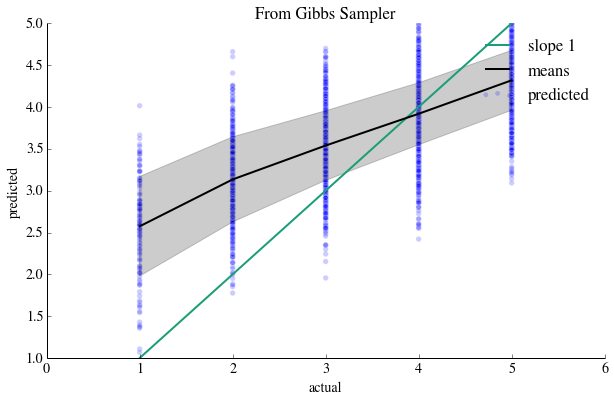your answer here The results from the latent factor model appear to be better-behaved than those from the KNN procedure (with negative similarities) since there are no extreme values. In terms of the non-extreme values from the KNN procedure, the latent factor model appears to make similar predictions to the KNN procedure when k = 3., Specifically, the average prediction line for each class is too compressed toward the total data mean of around 3.8, meaning that we are in the bias limit where we are pooling too much information between ratings. Thus, we are overpredicting low ratings and underpredicting high ratings. (If we compare to the KNN procedure with strictly positive weights, as in the homework addendum, we see again that the recommenders make comparable predictions, although the latent factor model again appears to fit slightly better than at both the bias or variance limits of the KNN procedure). There is also a bias-variance tradeoff here. In this case, it appears that we have proposed a model that is too simple (thus close to the bias limit) because of how the ratings are pulled in toward the data mean. In this case, proposing more latent factors increases the flexibility of the model, and thus moves us toward the variance limit. Thus, the plot suggests that we may want to reduce the bias at the cost of increasing variance, for example by considering a latent factor model with more factors (say,$L = 15\$) to obtain a better fit (see ADDENDUM below).

In :
gibbs_out = factor_gibbs(smalldf, 15, 1000, 0.1, 0.1)
burnin = 200
predicted=np.mean(gibbs_out['EY'][:,burnin:], axis=1)
compare_results(smalldf.stars.values, predicted, ylow=1, yhigh=5, title="From Gibbs Sampler")

0
100
200
300
400
500
600
700
800
900
fraction between -15 and 15 rating 1.0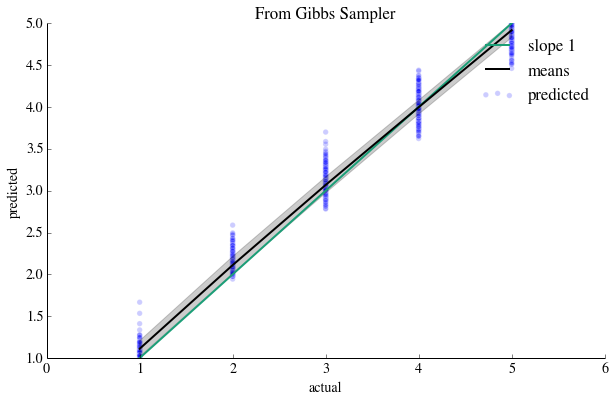The fit here looks much better, both in terms of tracking the green line and in terms of the within-group precision (as measured by the grey band). A model at the variance limit (that is, one that is extremely overfit) with low bias would have a mean line that tracks exactly with the green line and an almost non-existent gray area. To tell whether this plot represents simply a good prediction model or a one that is woefully overfit, we would need to look at out-of-sample data.

## Q4 Scaling Up¶

All our recommenders suffer from problems having to do with the fact that we subsetted an already sparse user-item matrix. The more items we have, the more items we may find in the vicinity of a given item, and thus we are likely to give a more robust average rating to the given item.

In this problem we shall use Amazon Elastic Map-Reduce to tackle the entire user-restaurant matrix. We shall do this in two parts: we'll use MRJob locally on your machine to on the smaller data set to calclate the pearson database, and then we'll tackle the entire data set on Amazon.

The larger set has 35000 users and 4500 items. Computing the 4500X4500 similarity matrix on one machine will be prohibitively expensive. Thus we'll adopt a strategy where we'll split the calculation over multiple machines using the map-reduce paradigm, with mappers and reducers working on multiple machines

Then we calculate the k-nearest neighbors in the 'space' of the user: this involves a database lookup and an iteration over the items a user has rated. Since the latter is usually not a very large number, this computation can be managed on a front end machine (even if storing the database will take a lot of memory).

We'll first create subset data frames, which have just those columns which we will send to the map-reduce. We'll also strip out the header and index of the frame. The reason for doing this is: unless we pre-populate the machines on Amazon with software, we can rely only on the regular python library, numpy, and scipy being there (and at python 2.6), and thus we will need to parse the csv file, line by line (mrjob uses hadoop's stream protocol and thus needs to be fed line by line).

In :
subsetoffull=fulldf[['user_id','business_id', 'stars','business_avg','user_avg']]


### Running mrjob locally¶

mrjob scripts cannot be run from the ipython notebook, as they fork themselves on execution. Thus you must write the code for mrjob in a separate file which you must submit along with this homework, in the same folder as the python notebook file.

If you have not done so already (you were supposed to do this as part of HW 0), you will first need to install mrjob. The appropriate equivalent of the following incantation should do the job:

~/anaconda/bin/pip install mrjob



To familiarize yourself with the structure of an mrjob script, please read this . Run the examples in that document to familiarize yourself with mrjob.

The kind of script you will be writing is in the section "Writing your second job" in that document.

All mrjob tasks use the map-reduce strategy to divide up computation across computers. You should work through the mrjob tutorial to gain familiarity with this, but we’ll also outline the basic process here:

1. During the first map step, mrjob calls a mapper function with a key (which for the first step is None), and a value (which for the first step is a line of data from an input file). This function does whatever it wants with this data, and yields a key and value. The key is used in step 2 to gather up the values from all the different mappers into groups

2. mrjob collects the outputs from all the mappers, and gathers them into subsets with the same key value (this is similar to what pandas.groupby does). It passes each of these subsets to a reducer (or “collector”) function, whose job is to synthesize this list of grouped data into something useful (e.g., computing the mean of all the inputs). It then yields the key and reduced value.

3. If there are any additional steps, mrjob feeds each output from a reducer function in step 2 to the next mapper. Otherwise, it prints the output.

The point behind map-reduce is to agree upon a common framework to split up a large computational job into smaller tasks. mrjob then has a lot of freedom to organize how these tasks run in parallel, on many machines

4.1 Write a MRJOB script, called computesim.py. The object of this script is to take a csv file and return a tuple (rho, n_common) as calculate_similarity for pairs of restaurants. See skeleton.py below for the SPEC of this file. Your job is to fill in those methods. You MUST use this skeleton.

This script is to be run like so (substitute your own operating system's call):

~/anaconda/bin/python computesim.py subset-small.csv > output.small.local.txt



Thus, when the script below is run in this fashion, mrjob will read the data line-by-line from subset-small.csv, and pass it to the first "step".

#### Algorithm to calculate pearson similarities¶

Here is the description of the algorithm for RestaurantSimilarities.

Your code will have two steps. Each step will have a mapper and a reducer. These are described in turn here:

1. line_mapper will split the line, yielding the user_id as key, and the rest as value. This method's implementation is provided for you.

2. users_items_collector is a reducer. It is passed ALL mapper outputs corresponding to a particular user_id. Put these emissions into a list, and re-emit the user_id with this list.

3. pair_items_mapper takes the user_id and the list. It dosent do anything with the user_id, however, it takes every combination (thus len(list) choose 2) of 2 business_ids from the passed on list (see combinations in itertools in the python documentation) and sends on the remaining information keyed on the tuple (restaurant1, restaurant2). Be sure to handle the case where the restaurant id's are flipped: include them somehow under the same key.

4. calc_sim_collector is passed ALL sent on list information for the pair of restaurants that was emitted in the previous step. Note that thse will come from different user_ids. This sort of collection is key to this style of programming. This list information should now correspond to all the common support of the two restaurants. Use this information to calculate this common support and the pearson similarity. Return the aforementioned tuple by yielding it keyed by the tuple of restaurants. This information will be sent to the output file. The output keys and values will both be in JSON format, separated by a tab.

The output should be saved in a file via redirection as output.small.local.txt

#### Skeleton File for this problem¶

You can access it here or just run the next cell to see it.

In :
from pygments import highlight
from pygments.lexers import PythonLexer
from pygments.formatters import HtmlFormatter
from IPython.display import HTML
import urllib
skelhtml=highlight(skelcode, PythonLexer(), HtmlFormatter())
HTML(skelhtml)

Out:
import numpy as np

from mrjob.job import MRJob
from itertools import combinations, permutations

from scipy.stats.stats import pearsonr

class RestaurantSimilarities(MRJob):

def steps(self):
"the steps in the map-reduce process"
thesteps = [
self.mr(mapper=self.line_mapper, reducer=self.users_items_collector),
self.mr(mapper=self.pair_items_mapper, reducer=self.calc_sim_collector)
]
return thesteps

def line_mapper(self,_,line):
"this is the complete implementation"

def users_items_collector(self, user_id, values):
"""
#iterate over the list of tuples yielded in the previous mapper
#and append them to an array of rating information
"""
pass

def pair_items_mapper(self, user_id, values):
"""
ignoring the user_id key, take all combinations of business pairs
and yield as key the pair id, and as value the pair rating information
"""

def calc_sim_collector(self, key, values):
"""
Pick up the information from the previous yield as shown. Compute
the pearson correlation and yield the final information as in the
last line here.
"""
(rest1, rest2), common_ratings = key, values
yield (rest1, rest2), (rho, n_common)

#Below MUST be there for things to work
if __name__ == '__main__':
RestaurantSimilarities.run()


#### Explanation for those funny yield keywords¶

The functions above “yield” values, and do not “return” them. They are generators. Here is an example:

In :
def upper_generator(words):
for word in words:
yield word.upper()

words = ['a', 'couple', 'of', 'words', 'to', 'process']

print upper_generator(words)
print list(upper_generator(words))
for u in upper_generator(words):
print u

<generator object upper_generator at 0x110b388c0>
['A', 'COUPLE', 'OF', 'WORDS', 'TO', 'PROCESS']
A
COUPLE
OF
WORDS
TO
PROCESS


You can read more here. Also see Thu Oct 17th's class video for information about classes and generators.

Include computesim.py in your submission in the same folder as the notebook. Uncommenting and running the following cell should output your code in here.

In :
thecode = open("computesim.py").read()
thehtml=highlight(thecode, PythonLexer(), HtmlFormatter())
HTML(thehtml)

Out:
import numpy as np

from mrjob.job import MRJob
from itertools import combinations, permutations
from math import sqrt

from scipy.stats.stats import pearsonr

class RestaurantSimilarities(MRJob):

def steps(self):
thesteps = [
self.mr(mapper=self.line_mapper, reducer=self.users_items_collector),
self.mr(mapper=self.pair_items_mapper, reducer=self.calc_sim_collector)
]
return thesteps

def line_mapper(self,_,line):

def users_items_collector(self, user_id, values):
ratings=[]
yield user_id, ratings

def pair_items_mapper(self, user_id, values):
ratings = values
for biz1tuple, biz2tuple in combinations(ratings, 2):
biz1, biz1r=biz1tuple
biz2, biz2r=biz2tuple
if biz1 <= biz2 :
yield (biz1, biz2), (biz1r, biz2r)
else:
yield (biz2, biz1), (biz2r, biz1r)

def calc_sim_collector(self, key, values):
(rest1, rest2), common_ratings = key, values
diff1=[]
diff2=[]
n_common=0

for rt1, rt2 in common_ratings:
diff1.append(float(rt1)-float(rt1))
diff2.append(float(rt2)-float(rt2))
n_common=n_common+1
if n_common==0:
rho=0.
else:
rho=pearsonr(diff1, diff2)
if np.isnan(rho):
rho=0.
yield (rest1, rest2), (rho, n_common)

#Below MUST be there for things to work!
if __name__ == '__main__':
RestaurantSimilarities.run()


#### Checking the results¶

Let us load the data from the file

In :
output_small_local=[[json.loads(j) for j in line.strip().split("\t")] for line in open("./output.small.local.txt")]
output_small_local

Out:
[[u'-4A5xmN21zi_TXnUESauUQ', u'-AAig9FG0s8gYE4f8GfowQ'],
[0.384365693729571, 5]]

We will Implement a function make_database_from_pairs which takes a dataframe of restaurants smalldf and the output parsed in the previous command to create the database like before. By the nature of the map-reduce algorithms these only contain those restaurant pairs with common support. The Database constructor initializes the remaining similarities to 0.

The function will take the dataframe and bizpairs obtained by parsing the EMR output file which have the key of business pairs and value the pair of pearson correlation and n_common. It will return an instance of the Database class.

This function will take a long time to run on large data sets.

In :
def make_database_from_pairs(df, bizpairs):
"""
make the database from the pairs returned from mrjob.
df is the dataframe, smalldf or fulldf.
bizpairs are a list of elements, each of which is a list of two
lists. The first of these lists has the two business id's, while
the second has the similarity and the common support
Returns an instance of the Database class.
"""
dbase=Database(df)
cache={}
for bp,corrs in bizpairs:
b1,b2=bp
i1=dbase.uniquebizids[b1]
i2=dbase.uniquebizids[b2]
sim,nsup=corrs
dbase.database_sim[i1][i2]=sim
dbase.database_sim[i2][i1]=sim
dbase.database_sup[i1][i2]=nsup
dbase.database_sup[i2][i1]=nsup
if cache.has_key(b1):
nsup1=cache[b1]
else:
cache[b1]=nsup1
if cache.has_key(b2):
nsup2=cache[b2]
else:
cache[b2]=nsup2
dbase.database_sim[i1][i1]=1.0
dbase.database_sim[i2][i2]=1.0
dbase.database_sup[i1][i1]=nsup1
dbase.database_sup[i2][i2]=nsup2
return dbase


We will store the output in variable db_mrjob_local.

In :
db_mrjob_local=make_database_from_pairs(smalldf, output_small_local)


We print a pair to see that our answers are identical.

In :
print db.get("zruUQvFySeXyEd7_rQixBg", "z3yFuLVrmH-3RJruPEMYKw")
print db_mrjob_local.get("zruUQvFySeXyEd7_rQixBg", "z3yFuLVrmH-3RJruPEMYKw")

(0.39904554525734559, 7)
(0.39904554525734542, 7)


4.2 Lets test that our results are overall the same as before

In :
sums=0.
count=0
for k in db.uniquebizids.keys():
for k2 in db.uniquebizids.keys():
count=count+1
sums=sums+db.get(k,k2)-db_mrjob_local.get(k,k2)
print sums, count

-8.65973959208e-15 29584


### Running on Amazon Elastic Map Reduce(EMR)¶

At this point, we shall shift to running on Amazon EMR.

Read this document for instructions on how to set yourself up on Amazon.

#### Reproduce the results with the smaller file on EMR¶

Test the smaller file and make sure it has the same results. For example, you could use the incantation:

~/anaconda/bin/python computesim.py -r emr --num-ec2-instances 2 subset-small.csv > output.small.emr.txt



You do NOT need to submit any results from that exploration to us.

Important: Please always make sure that your code is bug free, before actually submitting it to amazon. Try to run the job locally first and see if it produces the desired result. Then, if this worked, you are ready to proceed to the cloud. The homework problems are small and your free credit should provide you with a lot of room for running and testing on Amazon. However, it is your responsibility to make sure the jobs terminate properly and do not cause excessive costs.

You can always monitor your currently running jobs (in the US-East sector) using this overview at region US-EAST-1 of your MapReduce job flows.

#### Running the larger job¶

4.3 Run the script on the larger file subset-full.csv. Use between 4-8 instances on EMR on Amazon. Save the output in output.full.emr.txt. Your incantation will be something like:

~/anaconda/bin/python computesim.py -r emr --num-ec2-instances 5 subset-full.csv > output.full.emr.txt

You might elect to save the file on S3 and bring it over manually.

Try and think about what size job would be best to run on Amazon, given that there is a setup time. There is a way to persistently set up machines (the mrjob documentation provides the details), but then remember you will be billed for that setup and need to monitor it. However, a persistent setup might come useful for your projects.

Lets load the output in. CAUTION The next two cells will also take a lot of time to run and load.

In :
output_full_emr=[[json.loads(j) for j in l.strip().split("\t")] for l in open("./output.full.emr.txt")]


This function will take a very long time to run, on the order of 5 minutes or more, depending on your computer

In :
dbfull=make_database_from_pairs(fulldf, output_full_emr)


4.4 For testuserid, once again, print out the ratings using the bizs list as before. How have they changed with respect to Question 2? Why might this be?

In :
#your code here
for i in bizs:
print "========="
print biznamefromid(fulldf, i), i
print rating(fulldf, dbfull, i, testuserid, k=7, reg=3.)
u,a=get_other_ratings(i, testuserid, fulldf)
print "User Score:",u,"Avg score",a

for user Vern avg 3.58227848101
=========
Local Breeze GAPqG0WNBBidKeZTMpEZ-w
4.81062901451
User Score: 5 Avg score 3.86363636364
=========
Carly's Bistro zmFc8M-hS4uuyY0hklIpoQ
4.79797424984
User Score: 5 Avg score 3.65079365079
=========
Tee Pee Mexican Food soiGohHtWOltGeomkSxzEw
4.23927633965
User Score: 5 Avg score 3.13636363636
=========
District American Kitchen and Wine Bar 9ziO3NpoNTKHvIKCBFB_fQ
4.08622622369
User Score: 4 Avg score 3.575
=========
Los Reyes de la Torta bzDs0u8I-z231QVdIQWkrA
3.94718985123
User Score: 4 Avg score 4.24796747967


I copy here the results from the small set:

for user Vern avg 3.5652173913
----------------------------------
Local Breeze
Predicted Rating: 4.2280987611
Actual User Rating: 5 Avg Rating 4.0
----------------------------------
Carly's Bistro
Predicted Rating: 3.99008654065
Actual User Rating: 5 Avg Rating 3.5
----------------------------------
Tee Pee Mexican Food
Predicted Rating: 3.52640184162
Actual User Rating: 5 Avg Rating 3.04347826087
----------------------------------
District American Kitchen and Wine Bar
Predicted Rating: 3.80281696528
Actual User Rating: 4 Avg Rating 3.55263157895
----------------------------------
Los Reyes de la Torta
Predicted Rating: 3.41514298977
Actual User Rating: 4 Avg Rating 4.13157894737



One can see that the predicted rating is much closer to the actual one in the larger set. The reason for this: We are still working with user Vern from the previous set, so we are choosing a "prolific" user who is likely to have a dense neighborhood of restaurants in the larger set (by the choice of our cut to create the small set).

Note that if we evalusted our predictions on the entire data set, rather than cherry-picking users like Vern, our average prediction error may become worse. There is because the larger data set is more likely to be of higher sparsity, as we will include more users who rated very few restaurants.

4.5 Outline another step (in words) in the mrjob map-reduce class to implement a simple but scalable recommender of the global type that we did in Question 1.5 to 1.7.

MAP STEP:

def ranking_mapper(self, restaurants, values):
sim, n_common = values
rest1, rest2 = restaurants
if int(n_common) > 0:
yield (rest1, sim), (rest2, n_common)



REDUCE STEP:

    def top_similar_collector(self, key, values):
rest1, sim = key
for rest2, n_common in values:
yield None, (rest1, rest2, sim, n_common)



Note that by default mrjob does an alphanumeric sort on sim, which is not what we want. Indeed it is complicated, but possible to have this addition run locally (on mac/linux) you would need to specify the sort binary as sort -nr. On Hadoop, the parameters outlined here can be used. Of-course, you could do the final sorting on a front end machine anyway.

UPDATE: Brandon suggests an even simpler solution that will work both locally and on EMR, without having to use any hadoop sorting specifics. We change to:

MAP STEP:

def ranking_mapper(self, restaurants, values):
sim, n_common = values
rest1, rest2 = restaurants
if int(n_common) > 0:
yield (rest1), (sim, rest2, n_common)



REDUCE STEP:

    def top_similar_collector(self, key, values):
rest1 = key
for sim, rest2, n_common in sorted(values, reverse=True):
yield None, (rest1, rest2, sim, n_common)



Full code:

In :
thecode = open("computesim2.py").read()
thehtml=highlight(thecode, PythonLexer(), HtmlFormatter())
HTML(thehtml)

Out:
import numpy as np

from mrjob.job import MRJob
from itertools import combinations, permutations
from math import sqrt
import mrjob

from scipy.stats.stats import pearsonr

class RestaurantSimilarities(MRJob):

def steps(self):
thesteps = [
self.mr(mapper=self.line_mapper, reducer=self.users_items_collector),
self.mr(mapper=self.pair_items_mapper, reducer=self.calc_sim_collector),
self.mr(mapper=self.ranking_mapper, reducer=self.top_similar_collector)
]
return thesteps

def line_mapper(self,_,line):

def users_items_collector(self, user_id, values):
ratings=[]
yield user_id, ratings

def pair_items_mapper(self, user_id, values):
ratings = values
for biz1tuple, biz2tuple in combinations(ratings, 2):
biz1, biz1r=biz1tuple
biz2, biz2r=biz2tuple
if biz1 <= biz2 :
yield (biz1, biz2), (biz1r, biz2r)
else:
yield (biz2, biz1), (biz2r, biz1r)

def calc_sim_collector(self, key, values):
(rest1, rest2), common_ratings = key, values
diff1=[]
diff2=[]
n_common=0

for rt1, rt2 in common_ratings:
diff1.append(float(rt1)-float(rt1))
diff2.append(float(rt2)-float(rt2))
n_common=n_common+1
if n_common==0:
rho=0.
else:
rho=pearsonr(diff1, diff2)
if np.isnan(rho):
rho=0.
yield (rest1, rest2), (rho, n_common)

def ranking_mapper(self, restaurants, values):
sim, n_common = values
rest1, rest2 = restaurants
if int(n_common) > 0:
yield (rest1), (sim, rest2, n_common)

def top_similar_collector(self, key, values):
rest1 = key
for sim, rest2, n_common in sorted(values, reverse=True):
yield None, (rest1, rest2, sim, n_common)

#Below MUST be there for things to work!
if __name__ == '__main__':
RestaurantSimilarities.run()


To complete the recommender to the level of 1.7, we can now take the user's top restaurants, and repeat the process with the output of top_similar_collector, which could be stored in a hash table with restaurant keys and arrays of nearest neighbors.

### Submission Instructions:¶

Restart and run your notebook one last time (you do not have to rerun the Amazon EMR script computesim.py), to make sure the output from each cell is up to date. To submit your homework, create a folder named lastname_firstinitial_hw4 and place your solutions in the folder. Double check that the file is still called HW4.ipynb, and that it contains your code. Also include the computesim.py script and the output.small.local.txt data file. Do NOT include the data file output.full.emr.txt from the larger run (its huge, so we will check your answers to 4.4 instead). Compress the folder (please use .zip compression) and submit to the CS109 dropbox in the appropriate folder. If we cannot access your work because these directions are not followed correctly, we will not grade your work!

### FINI¶

You have developed all kinds of recommenders. We hope it was fun. Time constraints prevented us from going into model checking, but perhaps you would like to try that on your own. Or use S3 or a hosted database as a place to store sharded similarities. You might want to take a gander at Yelp's entire Phoenix dataset, or use the other attributes present in the data set. So many possibilities!